Скачать презентацию Totals Averages Marginals Part 2 Production

f10e9a799c2afd4ca023ea6ec0a31b13.ppt

• Количество слайдов: 80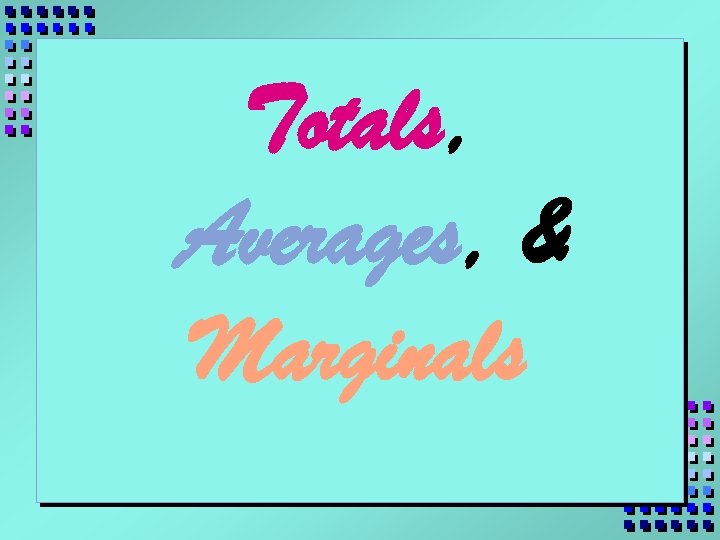Totals, Averages, & Marginals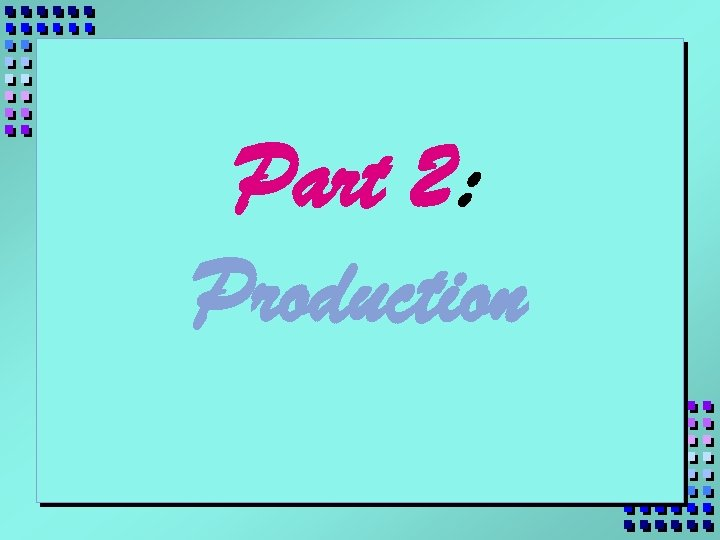Part 2: Production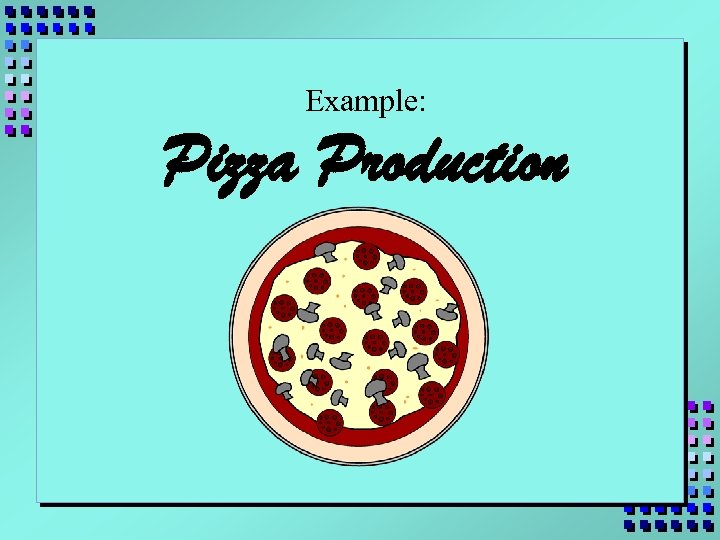Example: Pizza Production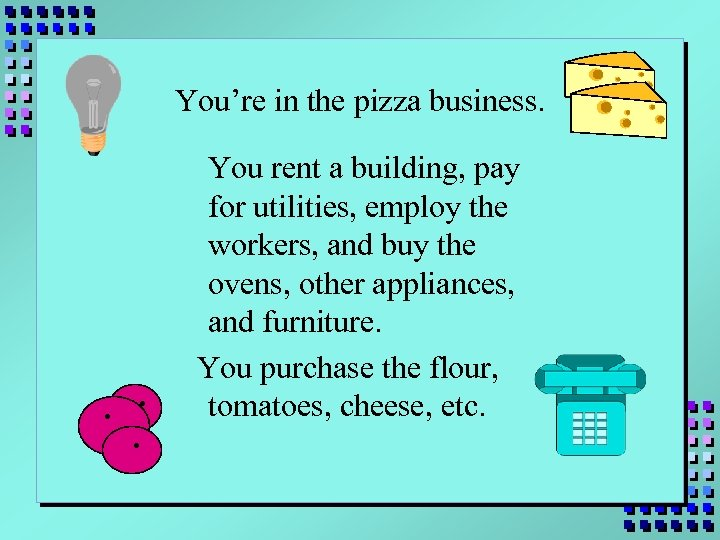You’re in the pizza business. . You rent a building, pay for utilities, employ the workers, and buy the ovens, other appliances, and furniture. You purchase the flour, tomatoes, cheese, etc.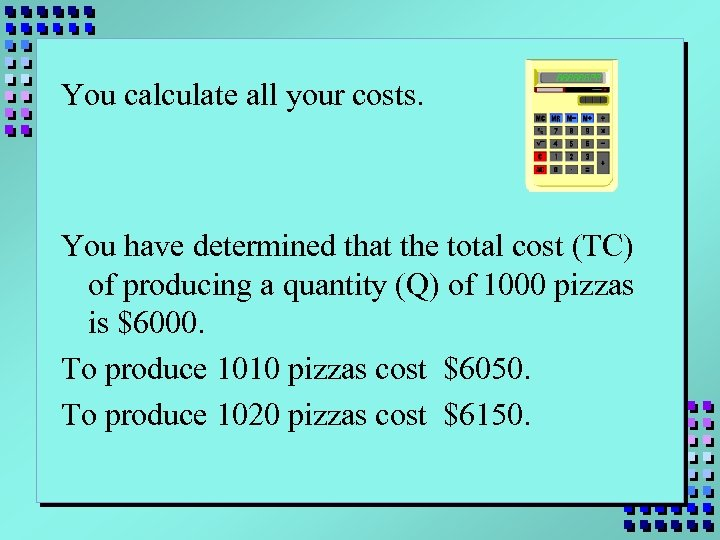You calculate all your costs. You have determined that the total cost (TC) of producing a quantity (Q) of 1000 pizzas is \$6000. To produce 1010 pizzas cost \$6050. To produce 1020 pizzas cost \$6150.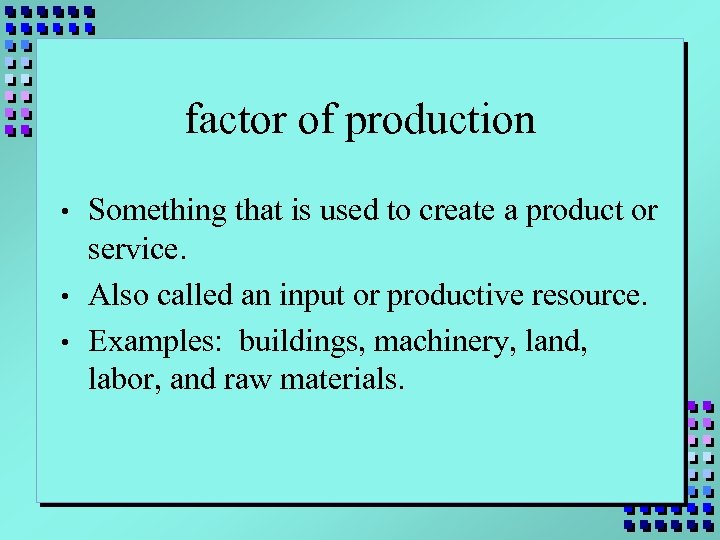factor of production • • • Something that is used to create a product or service. Also called an input or productive resource. Examples: buildings, machinery, land, labor, and raw materials.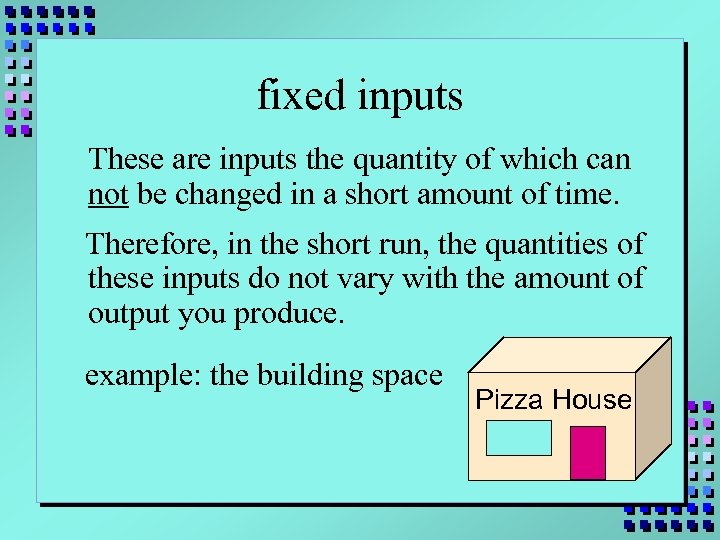fixed inputs These are inputs the quantity of which can not be changed in a short amount of time. Therefore, in the short run, the quantities of these inputs do not vary with the amount of output you produce. example: the building space Pizza House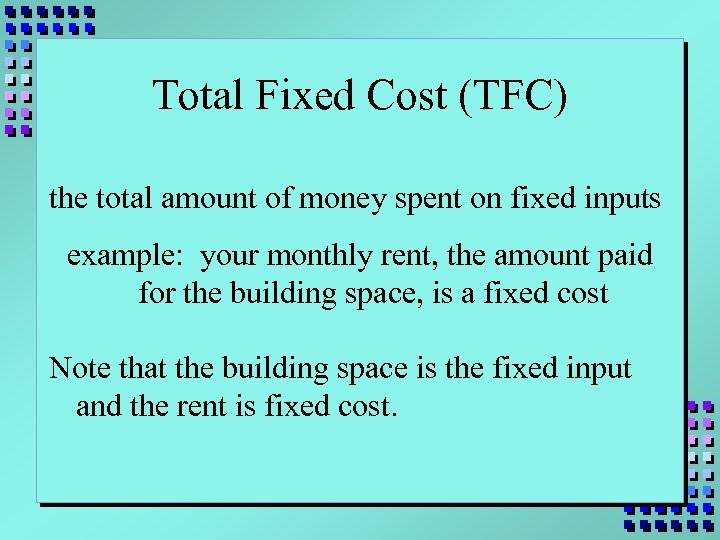Total Fixed Cost (TFC) the total amount of money spent on fixed inputs example: your monthly rent, the amount paid for the building space, is a fixed cost Note that the building space is the fixed input and the rent is fixed cost.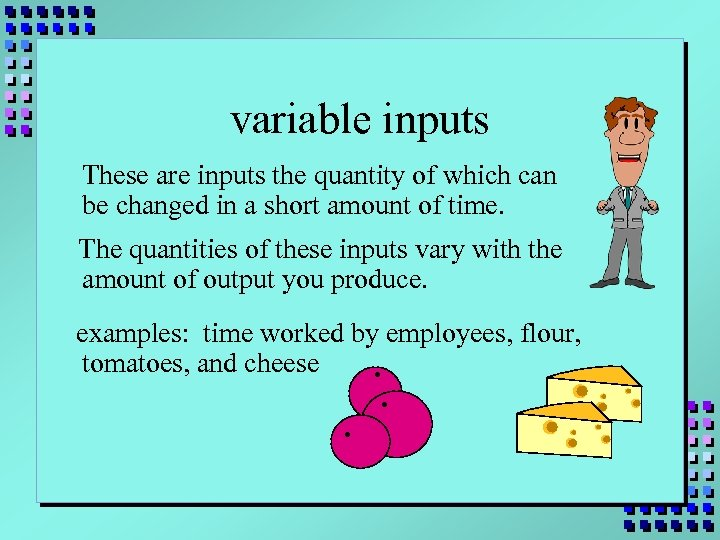variable inputs These are inputs the quantity of which can be changed in a short amount of time. The quantities of these inputs vary with the amount of output you produce. examples: time worked by employees, flour, tomatoes, and cheese. . .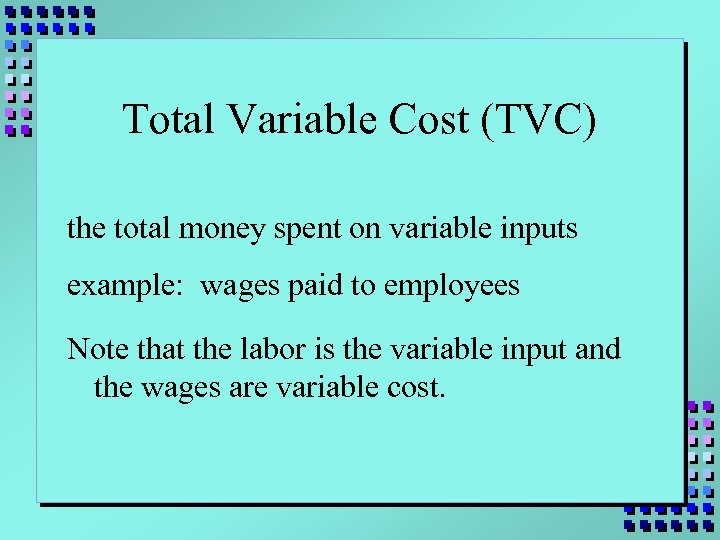Total Variable Cost (TVC) the total money spent on variable inputs example: wages paid to employees Note that the labor is the variable input and the wages are variable cost.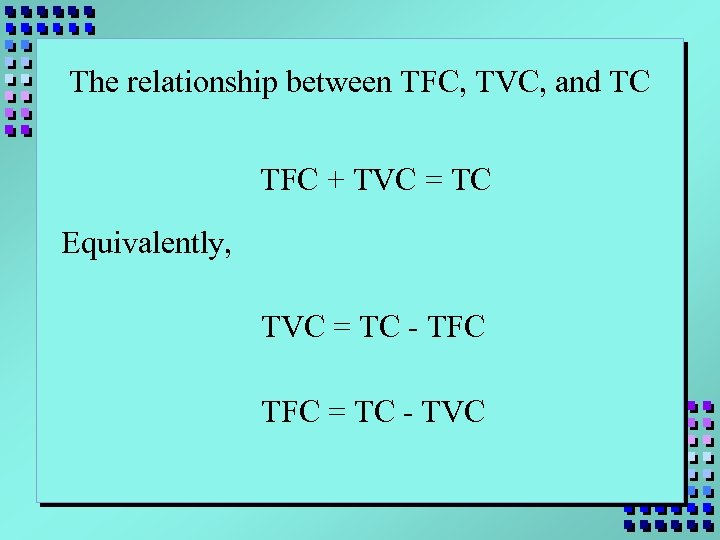The relationship between TFC, TVC, and TC TFC + TVC = TC Equivalently, TVC = TC - TFC = TC - TVC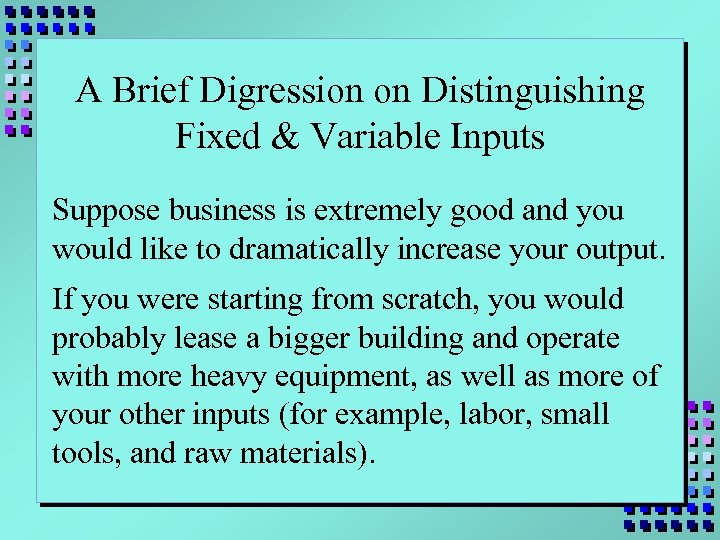A Brief Digression on Distinguishing Fixed & Variable Inputs Suppose business is extremely good and you would like to dramatically increase your output. If you were starting from scratch, you would probably lease a bigger building and operate with more heavy equipment, as well as more of your other inputs (for example, labor, small tools, and raw materials).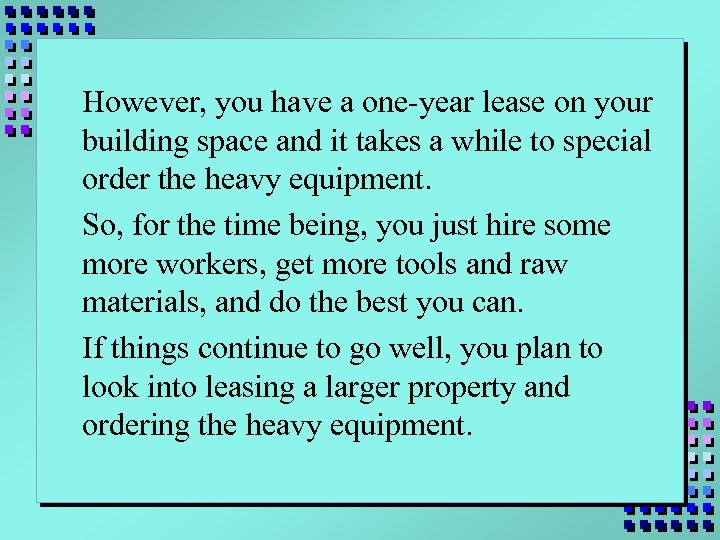However, you have a one-year lease on your building space and it takes a while to special order the heavy equipment. So, for the time being, you just hire some more workers, get more tools and raw materials, and do the best you can. If things continue to go well, you plan to look into leasing a larger property and ordering the heavy equipment.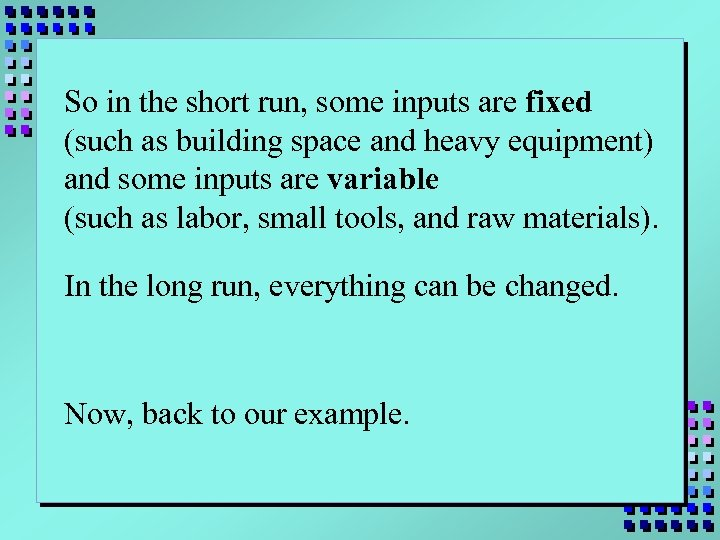So in the short run, some inputs are fixed (such as building space and heavy equipment) and some inputs are variable (such as labor, small tools, and raw materials). In the long run, everything can be changed. Now, back to our example.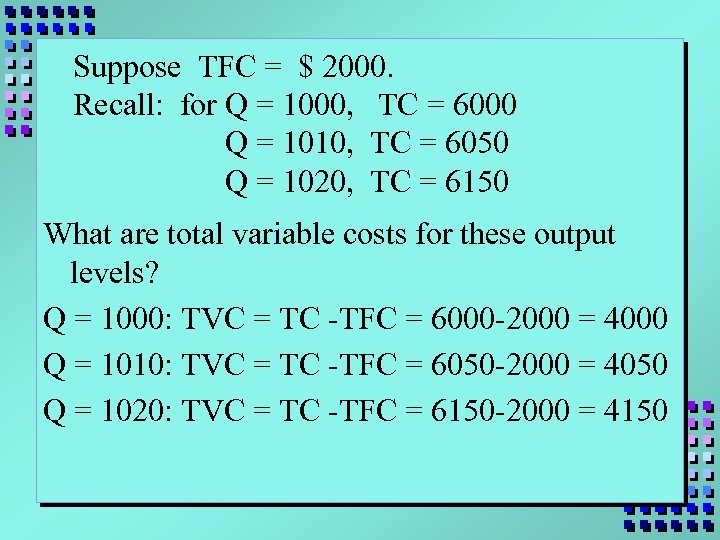Suppose TFC = \$ 2000. Recall: for Q = 1000, TC = 6000 Q = 1010, TC = 6050 Q = 1020, TC = 6150 What are total variable costs for these output levels? Q = 1000: TVC = TC -TFC = 6000 -2000 = 4000 Q = 1010: TVC = TC -TFC = 6050 -2000 = 4050 Q = 1020: TVC = TC -TFC = 6150 -2000 = 4150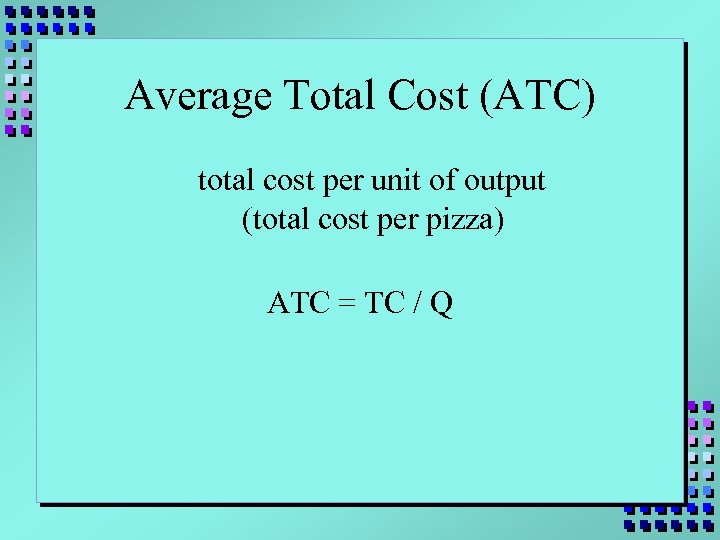Average Total Cost (ATC) total cost per unit of output (total cost per pizza) ATC = TC / Q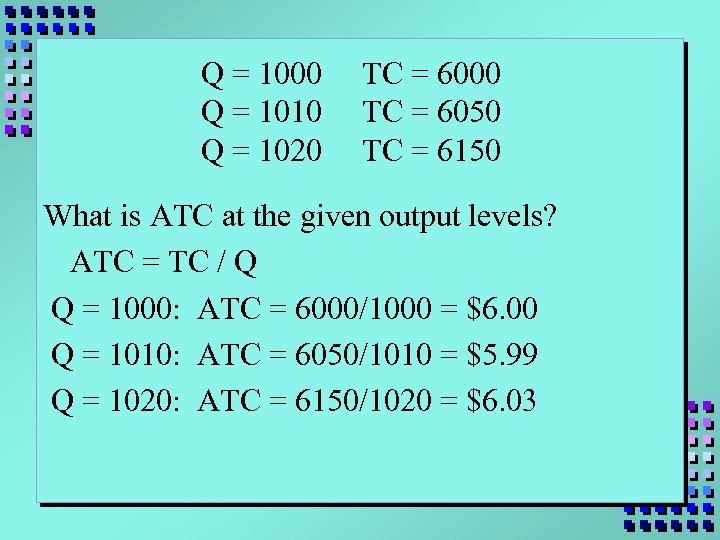Q = 1000 Q = 1010 Q = 1020 TC = 6000 TC = 6050 TC = 6150 What is ATC at the given output levels? ATC = TC / Q Q = 1000: ATC = 6000/1000 = \$6. 00 Q = 1010: ATC = 6050/1010 = \$5. 99 Q = 1020: ATC = 6150/1020 = \$6. 03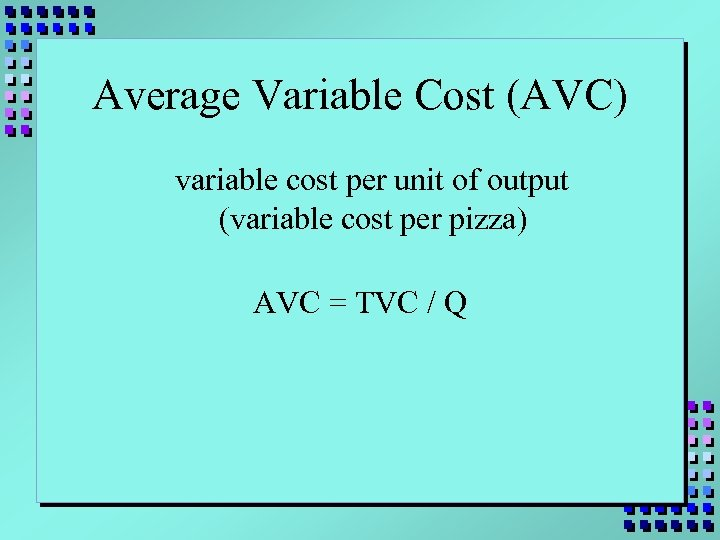Average Variable Cost (AVC) variable cost per unit of output (variable cost per pizza) AVC = TVC / Q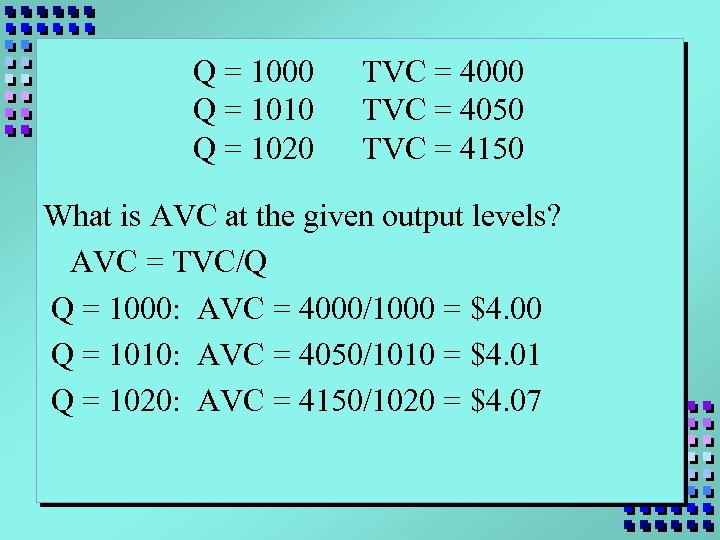Q = 1000 Q = 1010 Q = 1020 TVC = 4000 TVC = 4050 TVC = 4150 What is AVC at the given output levels? AVC = TVC/Q Q = 1000: AVC = 4000/1000 = \$4. 00 Q = 1010: AVC = 4050/1010 = \$4. 01 Q = 1020: AVC = 4150/1020 = \$4. 07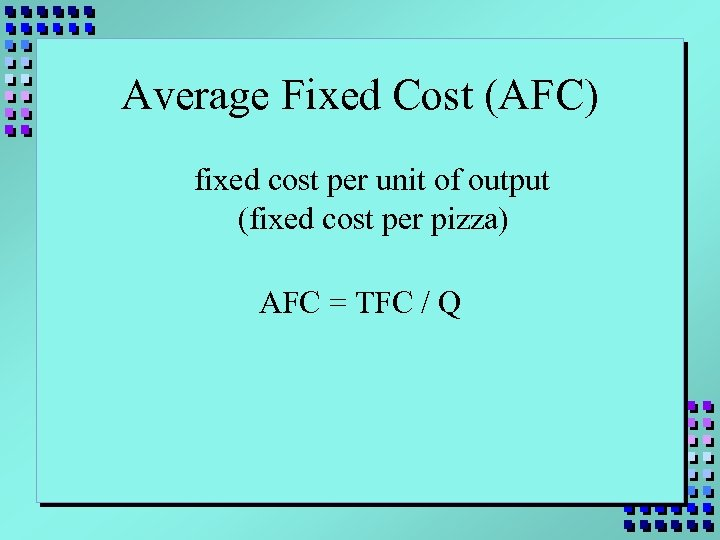Average Fixed Cost (AFC) fixed cost per unit of output (fixed cost per pizza) AFC = TFC / Q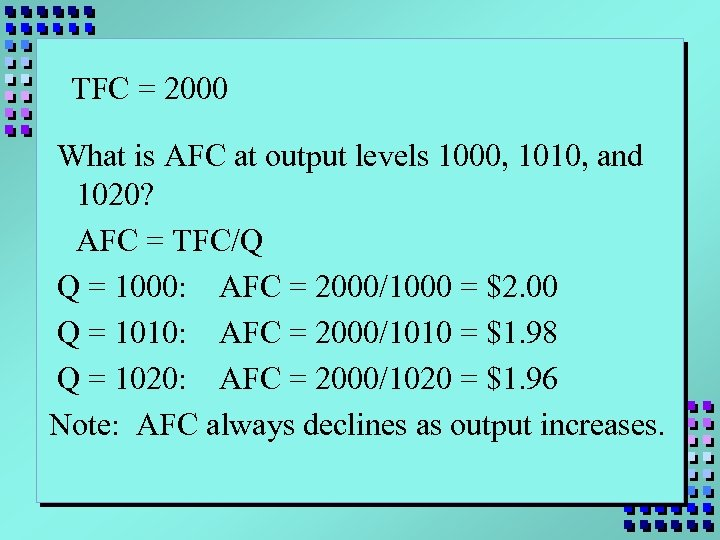TFC = 2000 What is AFC at output levels 1000, 1010, and 1020? AFC = TFC/Q Q = 1000: AFC = 2000/1000 = \$2. 00 Q = 1010: AFC = 2000/1010 = \$1. 98 Q = 1020: AFC = 2000/1020 = \$1. 96 Note: AFC always declines as output increases.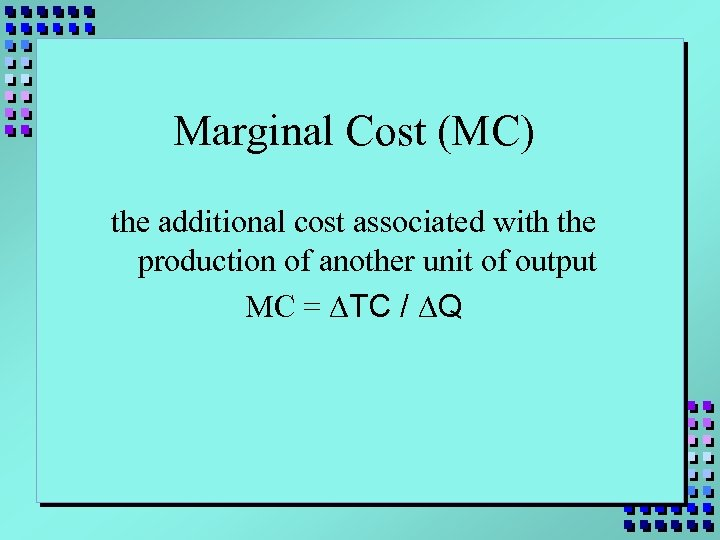Marginal Cost (MC) the additional cost associated with the production of another unit of output MC = DTC / DQ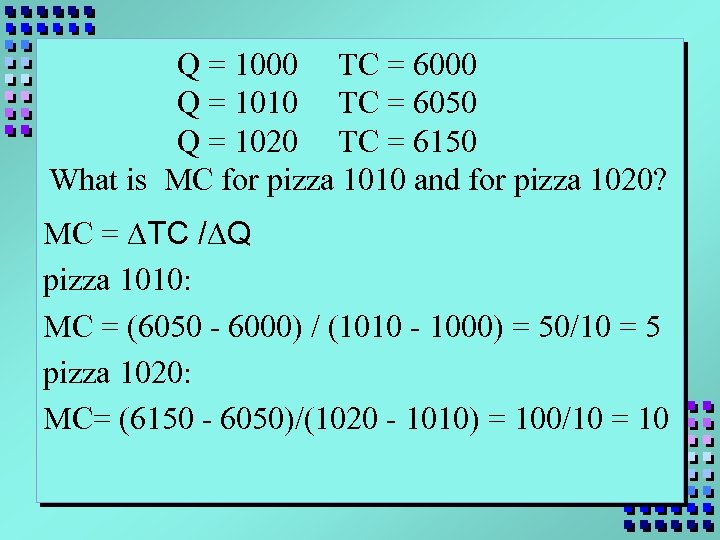Q = 1000 TC = 6000 Q = 1010 TC = 6050 Q = 1020 TC = 6150 What is MC for pizza 1010 and for pizza 1020? MC = DTC /DQ pizza 1010: MC = (6050 - 6000) / (1010 - 1000) = 50/10 = 5 pizza 1020: MC= (6150 - 6050)/(1020 - 1010) = 100/10 = 10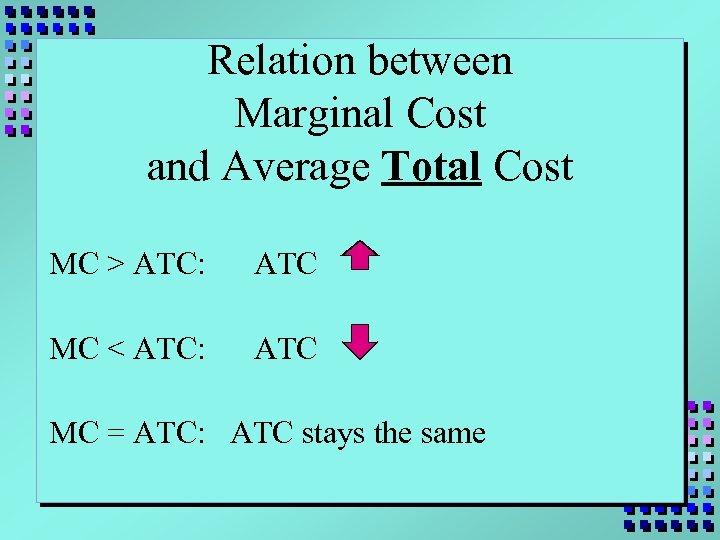Relation between Marginal Cost and Average Total Cost MC > ATC: ATC MC < ATC: ATC MC = ATC: ATC stays the same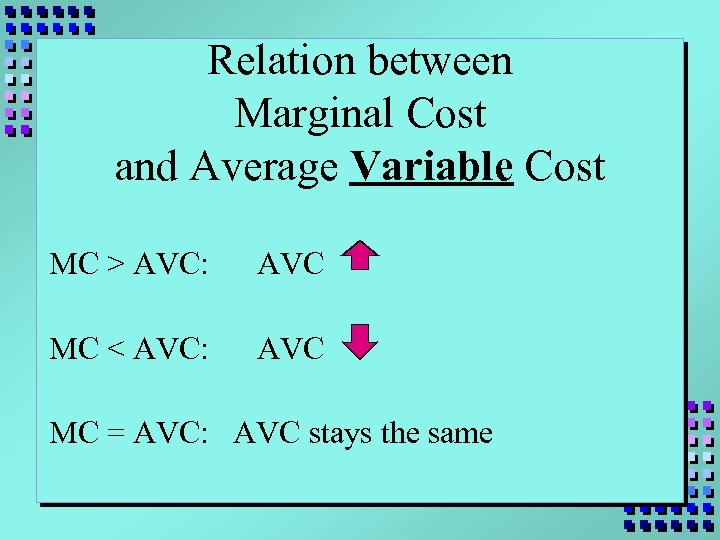Relation between Marginal Cost and Average Variable Cost MC > AVC: AVC MC < AVC: AVC MC = AVC: AVC stays the same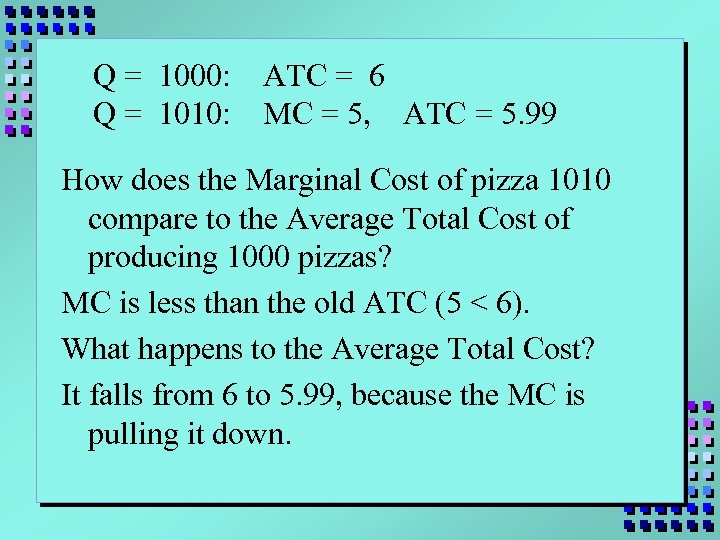Q = 1000: Q = 1010: ATC = 6 MC = 5, ATC = 5. 99 How does the Marginal Cost of pizza 1010 compare to the Average Total Cost of producing 1000 pizzas? MC is less than the old ATC (5 < 6). What happens to the Average Total Cost? It falls from 6 to 5. 99, because the MC is pulling it down.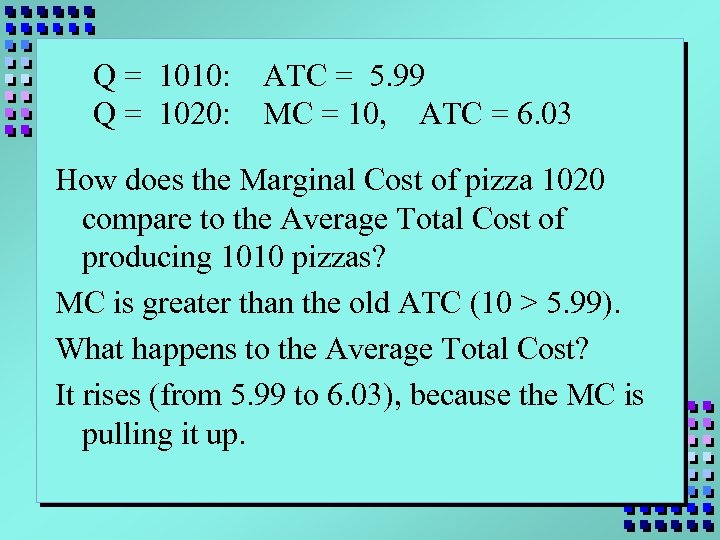Q = 1010: Q = 1020: ATC = 5. 99 MC = 10, ATC = 6. 03 How does the Marginal Cost of pizza 1020 compare to the Average Total Cost of producing 1010 pizzas? MC is greater than the old ATC (10 > 5. 99). What happens to the Average Total Cost? It rises (from 5. 99 to 6. 03), because the MC is pulling it up.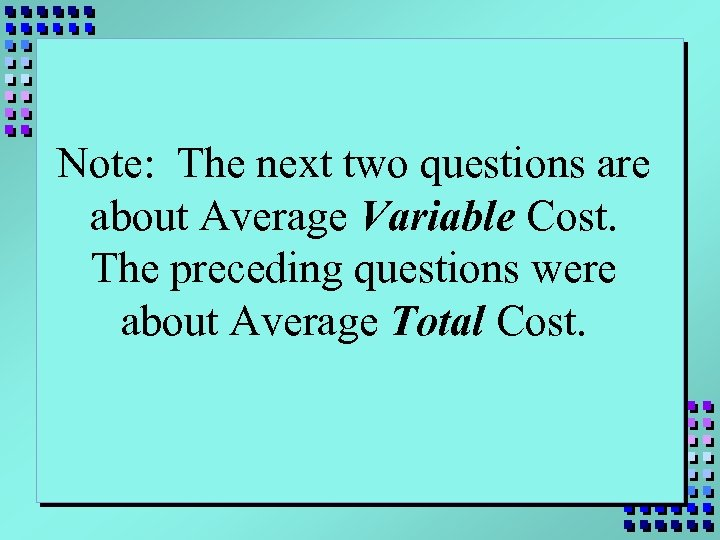Note: The next two questions are about Average Variable Cost. The preceding questions were about Average Total Cost.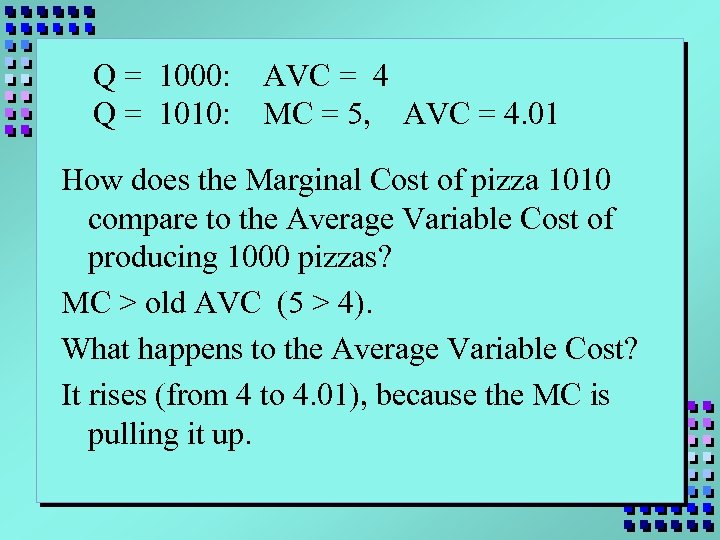Q = 1000: Q = 1010: AVC = 4 MC = 5, AVC = 4. 01 How does the Marginal Cost of pizza 1010 compare to the Average Variable Cost of producing 1000 pizzas? MC > old AVC (5 > 4). What happens to the Average Variable Cost? It rises (from 4 to 4. 01), because the MC is pulling it up.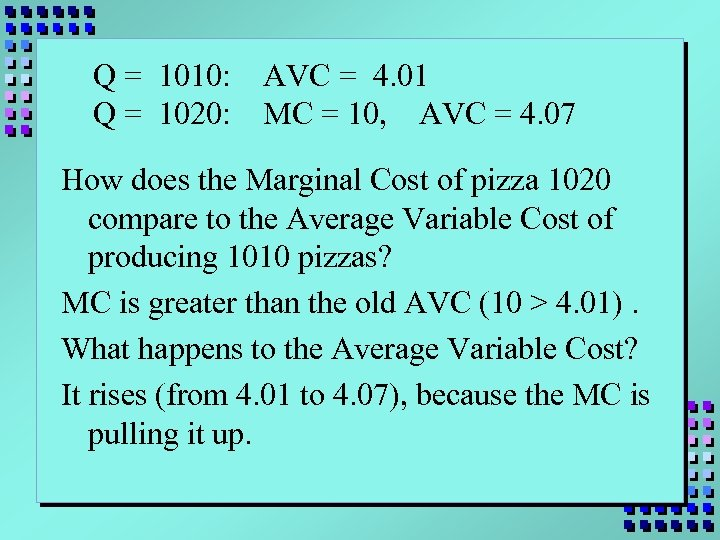Q = 1010: Q = 1020: AVC = 4. 01 MC = 10, AVC = 4. 07 How does the Marginal Cost of pizza 1020 compare to the Average Variable Cost of producing 1010 pizzas? MC is greater than the old AVC (10 > 4. 01). What happens to the Average Variable Cost? It rises (from 4. 01 to 4. 07), because the MC is pulling it up.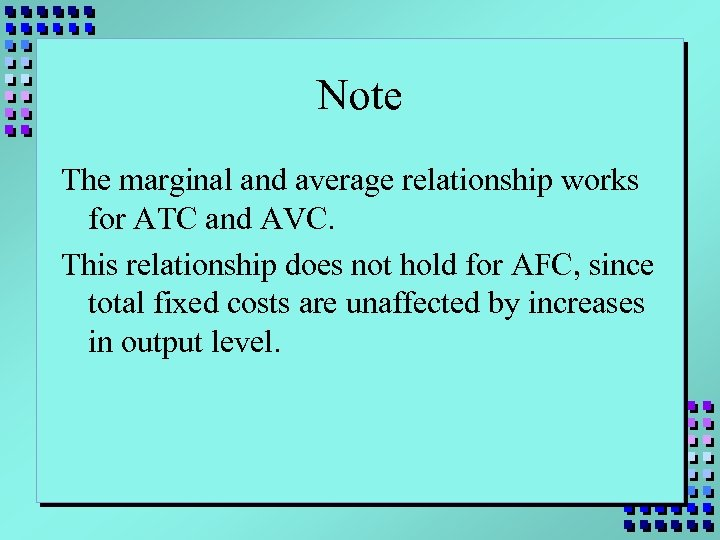Note The marginal and average relationship works for ATC and AVC. This relationship does not hold for AFC, since total fixed costs are unaffected by increases in output level.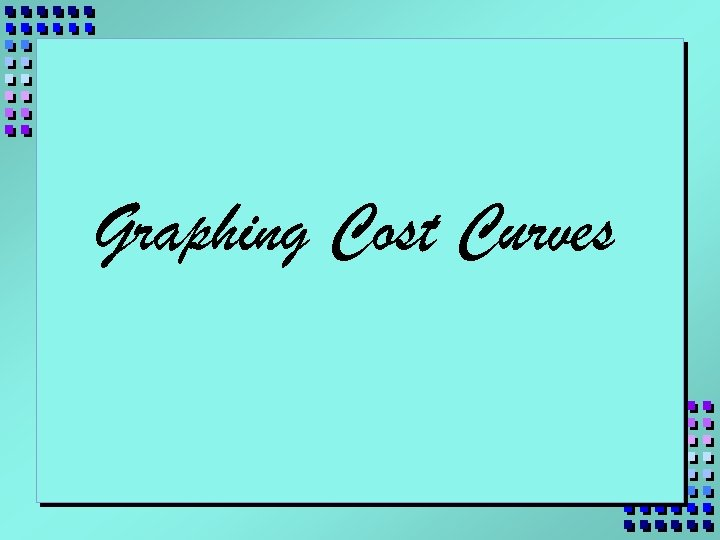Graphing Cost Curves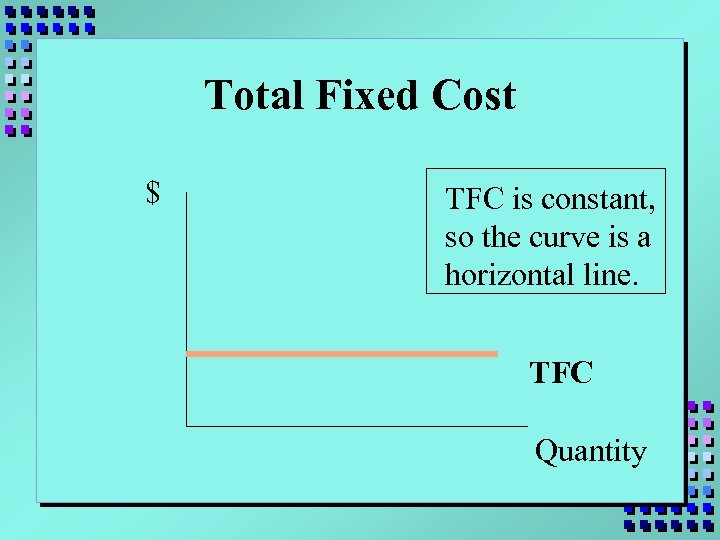Total Fixed Cost \$ TFC is constant, so the curve is a horizontal line. TFC Quantity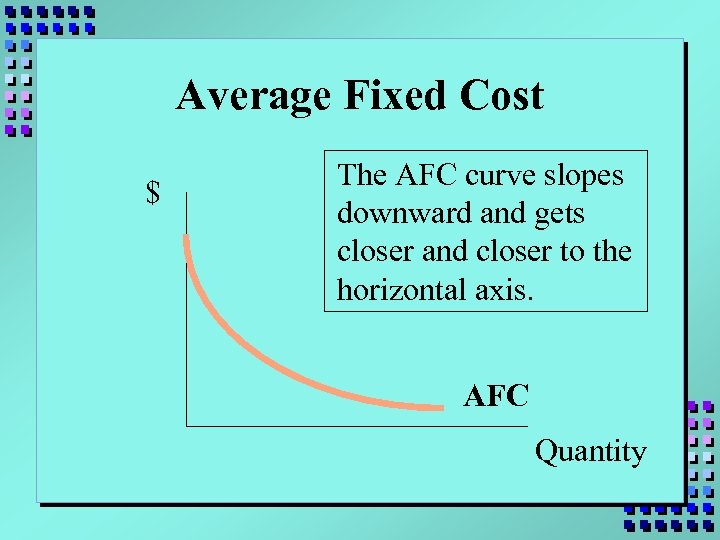Average Fixed Cost \$ The AFC curve slopes downward and gets closer and closer to the horizontal axis. AFC Quantity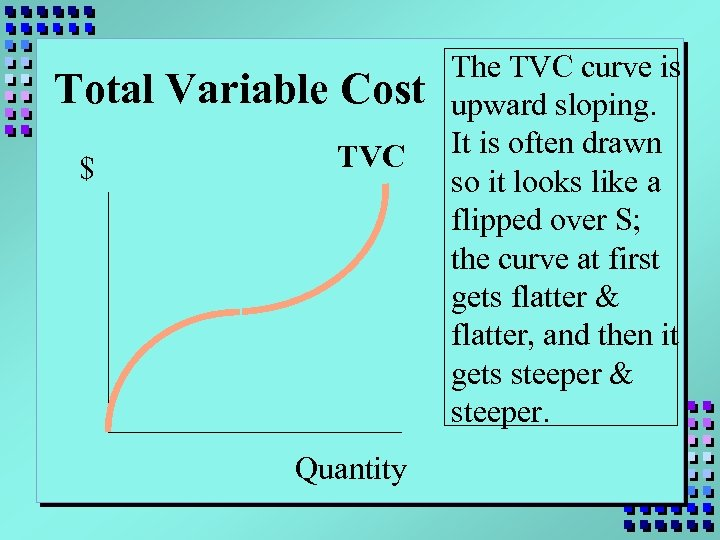Total Variable Cost \$ TVC Quantity The TVC curve is upward sloping. It is often drawn so it looks like a flipped over S; the curve at first gets flatter & flatter, and then it gets steeper & steeper.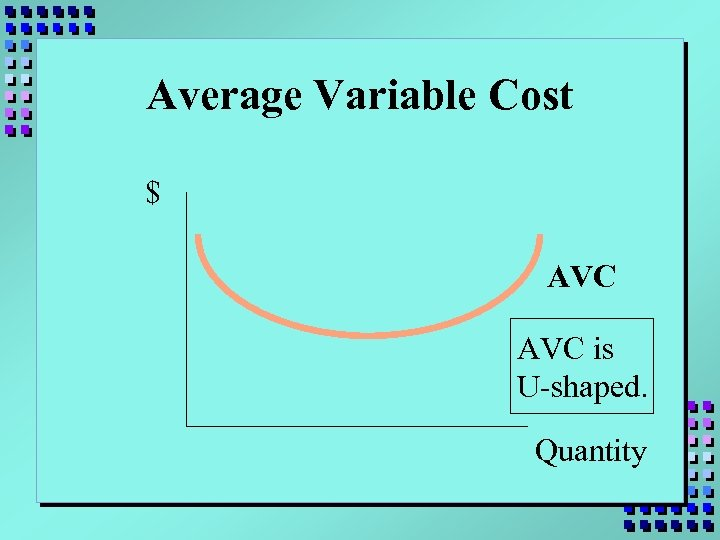Average Variable Cost \$ AVC is U-shaped. Quantity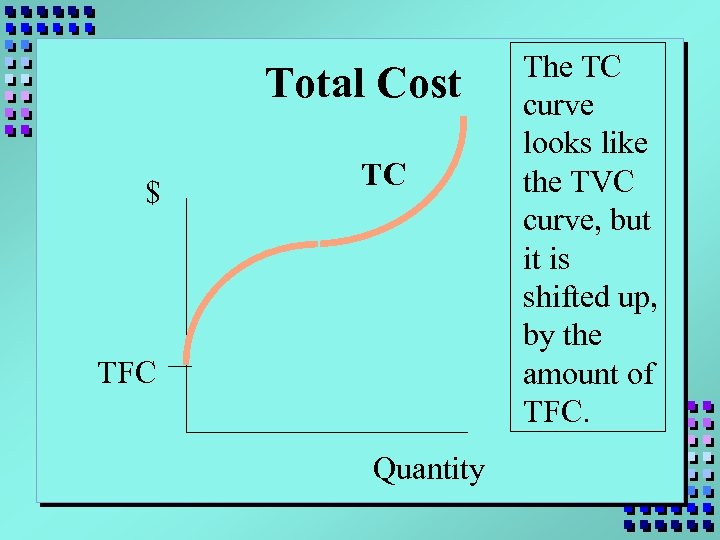Total Cost \$ TC TFC Quantity The TC curve looks like the TVC curve, but it is shifted up, by the amount of TFC.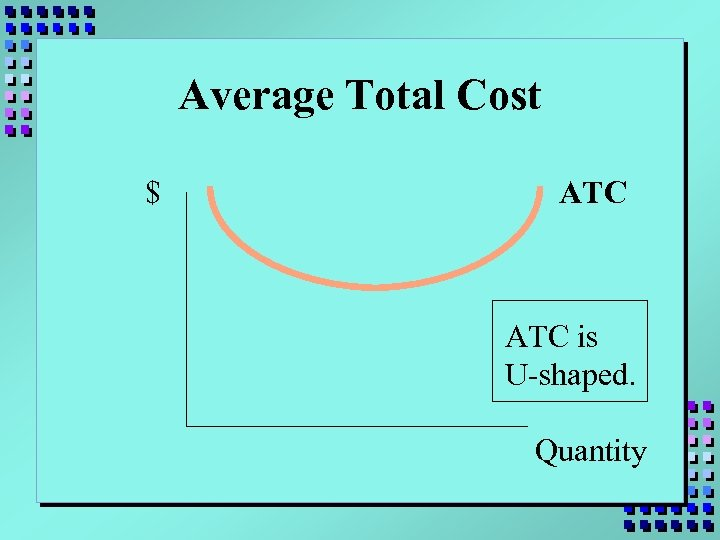Average Total Cost \$ ATC is U-shaped. Quantity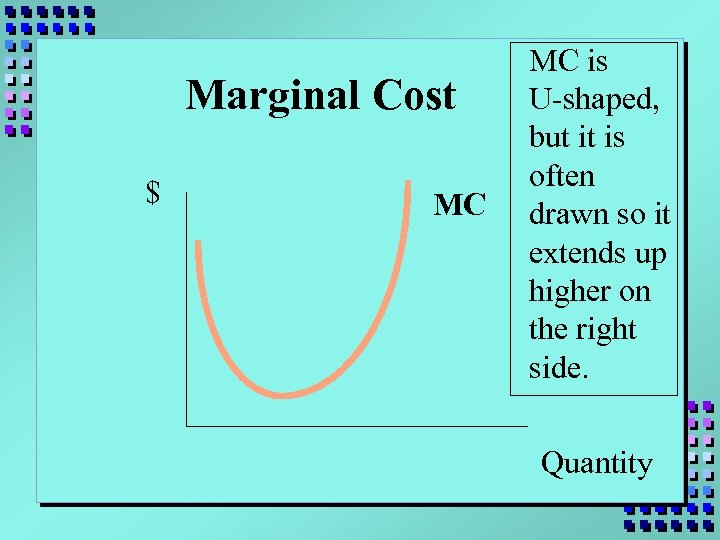Marginal Cost \$ MC MC is U-shaped, but it is often drawn so it extends up higher on the right side. Quantity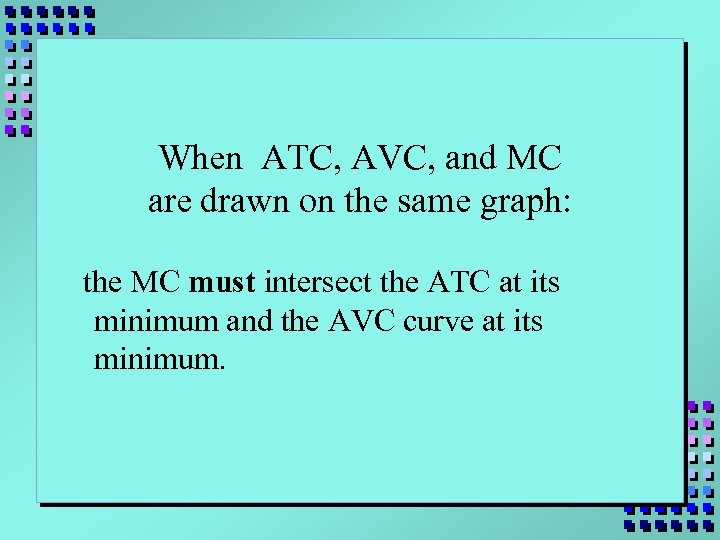When ATC, AVC, and MC are drawn on the same graph: the MC must intersect the ATC at its minimum and the AVC curve at its minimum.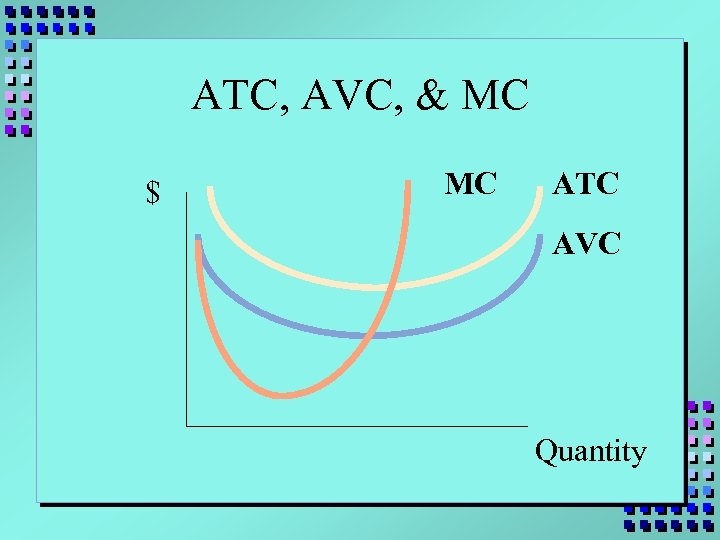ATC, AVC, & MC \$ MC ATC AVC Quantity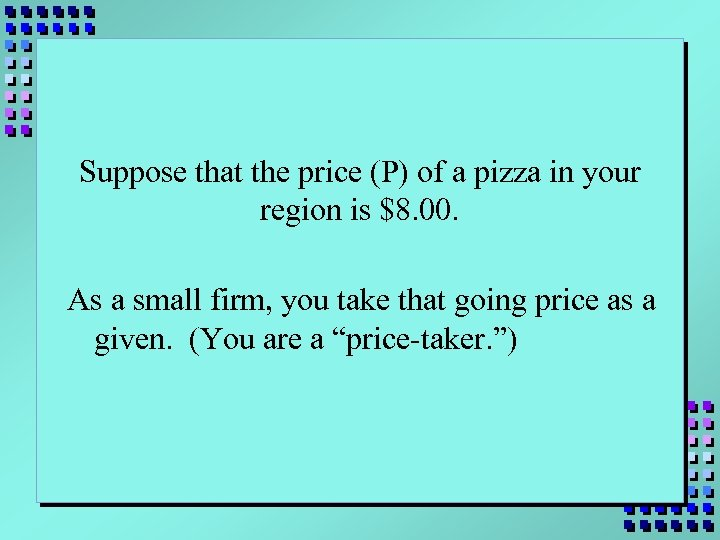Suppose that the price (P) of a pizza in your region is \$8. 00. As a small firm, you take that going price as a given. (You are a “price-taker. ”)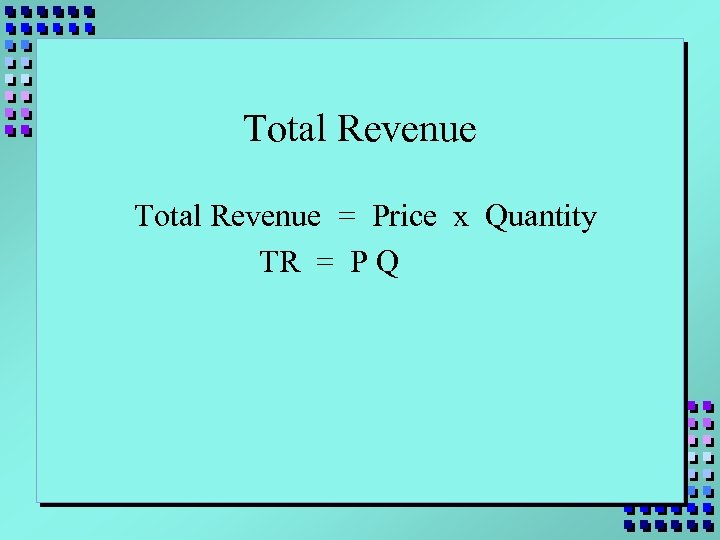Total Revenue = Price x Quantity TR = P Q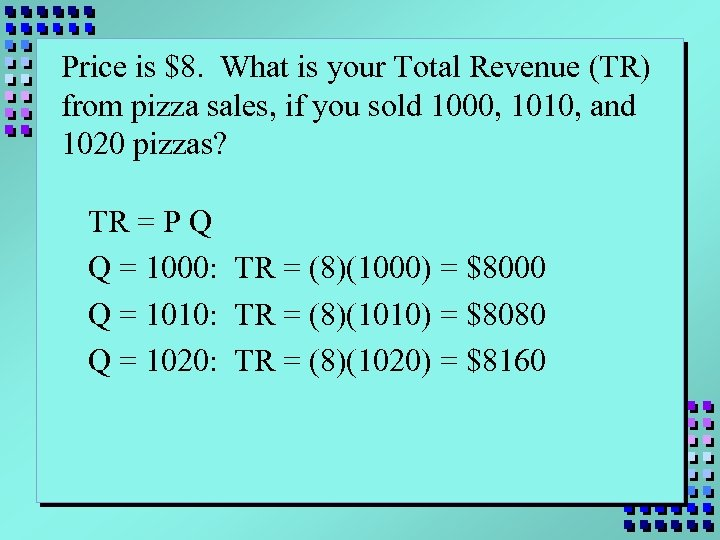Price is \$8. What is your Total Revenue (TR) from pizza sales, if you sold 1000, 1010, and 1020 pizzas? TR = P Q Q = 1000: TR = (8)(1000) = \$8000 Q = 1010: TR = (8)(1010) = \$8080 Q = 1020: TR = (8)(1020) = \$8160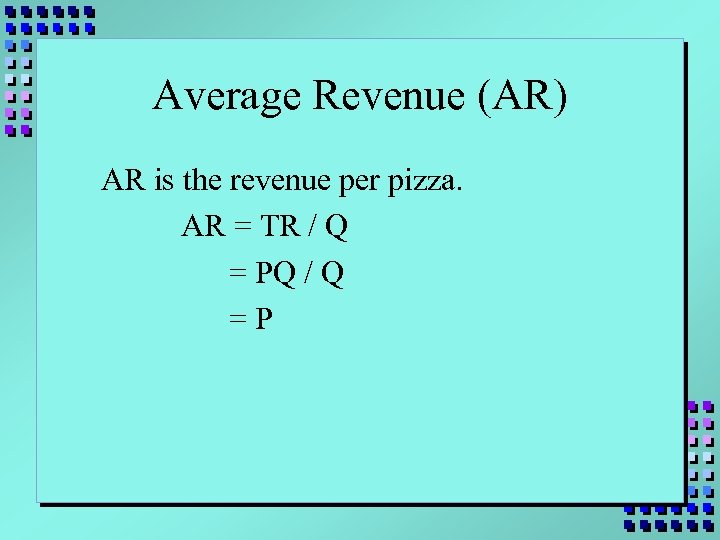Average Revenue (AR) AR is the revenue per pizza. AR = TR / Q = PQ / Q =P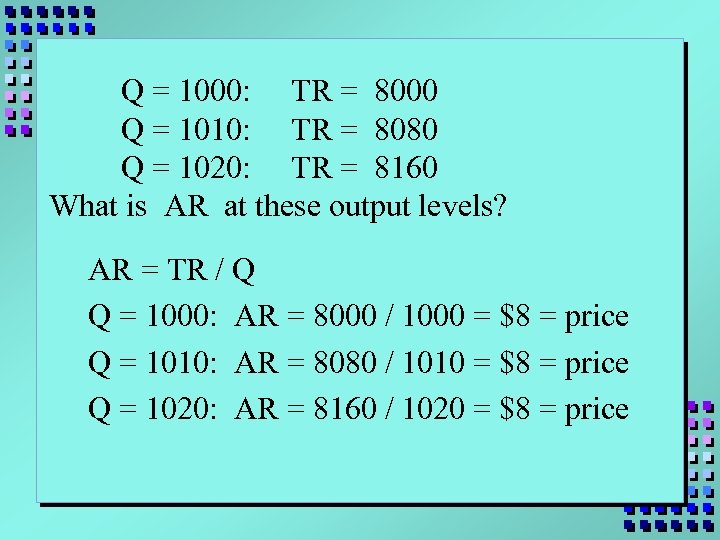Q = 1000: TR = 8000 Q = 1010: TR = 8080 Q = 1020: TR = 8160 What is AR at these output levels? AR = TR / Q Q = 1000: AR = 8000 / 1000 = \$8 = price Q = 1010: AR = 8080 / 1010 = \$8 = price Q = 1020: AR = 8160 / 1020 = \$8 = price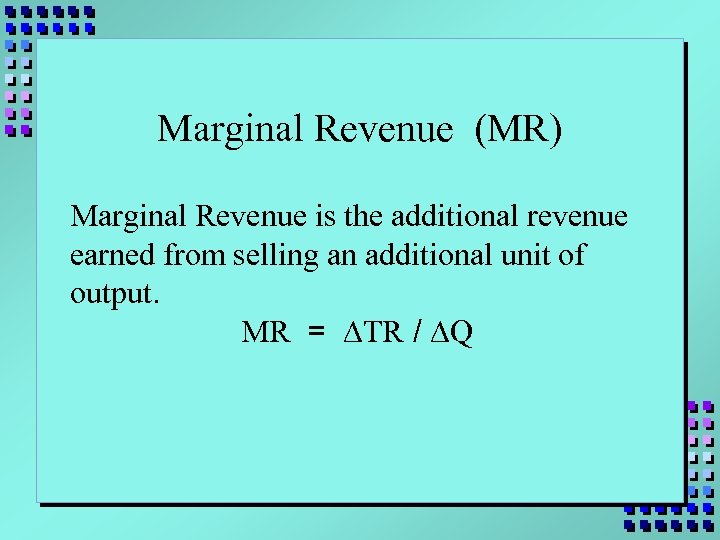Marginal Revenue (MR) Marginal Revenue is the additional revenue earned from selling an additional unit of output. MR = DTR / DQ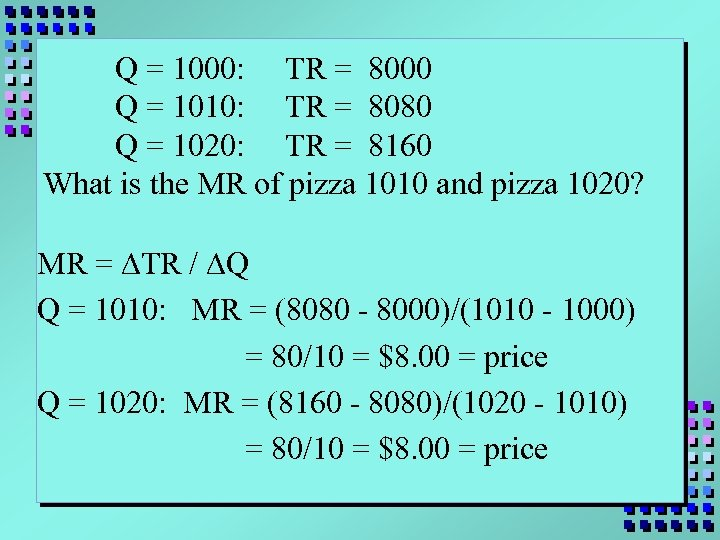Q = 1000: TR = 8000 Q = 1010: TR = 8080 Q = 1020: TR = 8160 What is the MR of pizza 1010 and pizza 1020? MR = DTR / DQ Q = 1010: MR = (8080 - 8000)/(1010 - 1000) = 80/10 = \$8. 00 = price Q = 1020: MR = (8160 - 8080)/(1020 - 1010) = 80/10 = \$8. 00 = price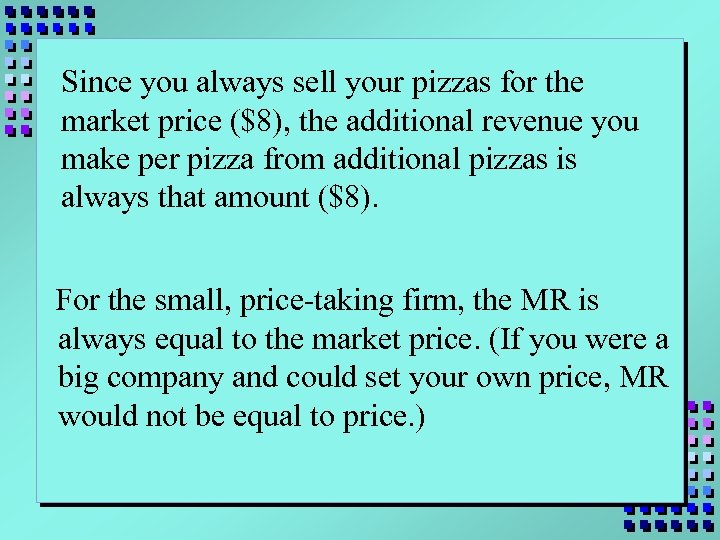Since you always sell your pizzas for the market price (\$8), the additional revenue you make per pizza from additional pizzas is always that amount (\$8). For the small, price-taking firm, the MR is always equal to the market price. (If you were a big company and could set your own price, MR would not be equal to price. )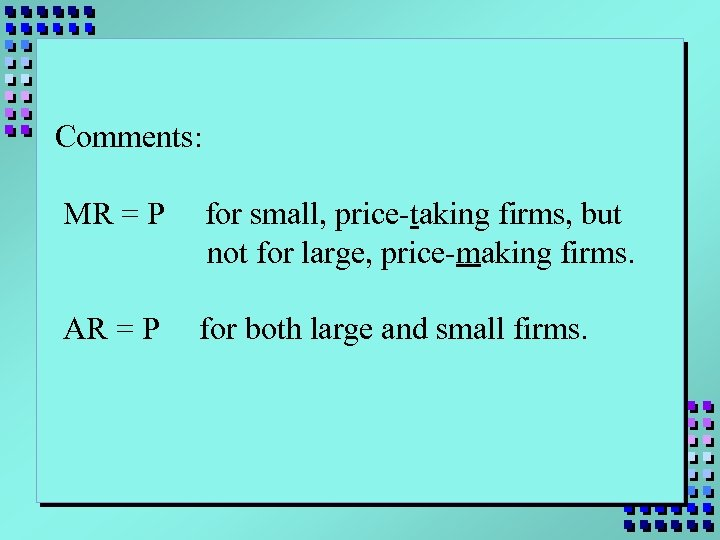Comments: MR = P for small, price-taking firms, but not for large, price-making firms. AR = P for both large and small firms.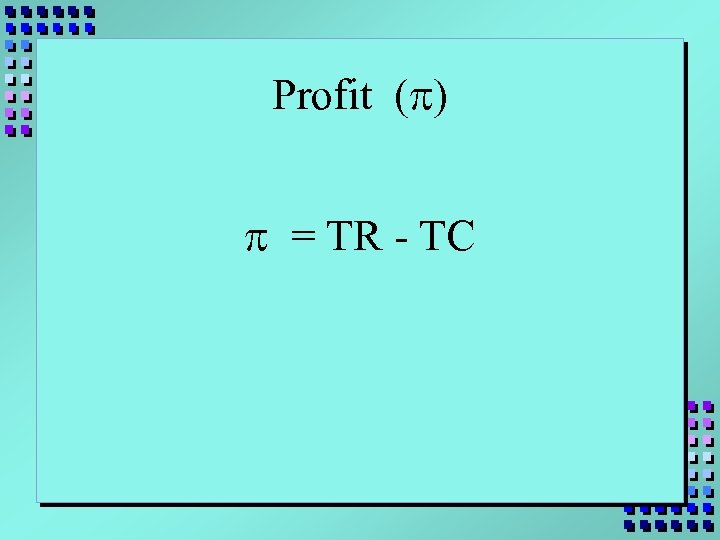Profit (p) p = TR - TC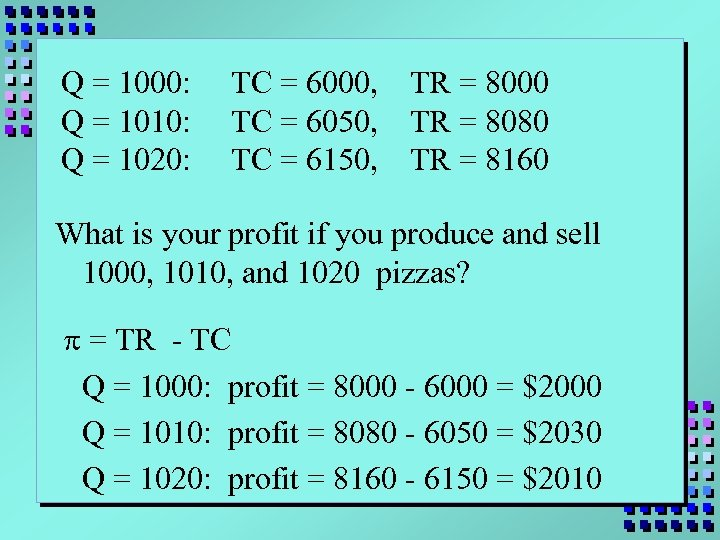Q = 1000: Q = 1010: Q = 1020: TC = 6000, TC = 6050, TC = 6150, TR = 8000 TR = 8080 TR = 8160 What is your profit if you produce and sell 1000, 1010, and 1020 pizzas? p = TR - TC Q = 1000: profit = 8000 - 6000 = \$2000 Q = 1010: profit = 8080 - 6050 = \$2030 Q = 1020: profit = 8160 - 6150 = \$2010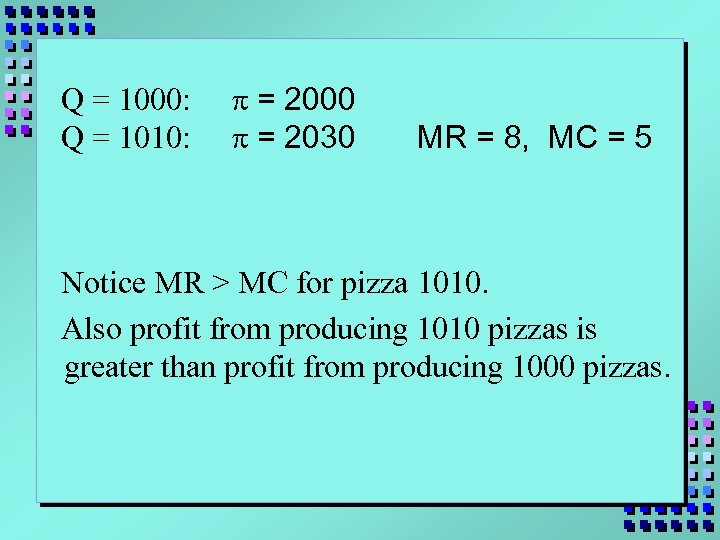Q = 1000: Q = 1010: p = 2000 p = 2030 MR = 8, MC = 5 Notice MR > MC for pizza 1010. Also profit from producing 1010 pizzas is greater than profit from producing 1000 pizzas.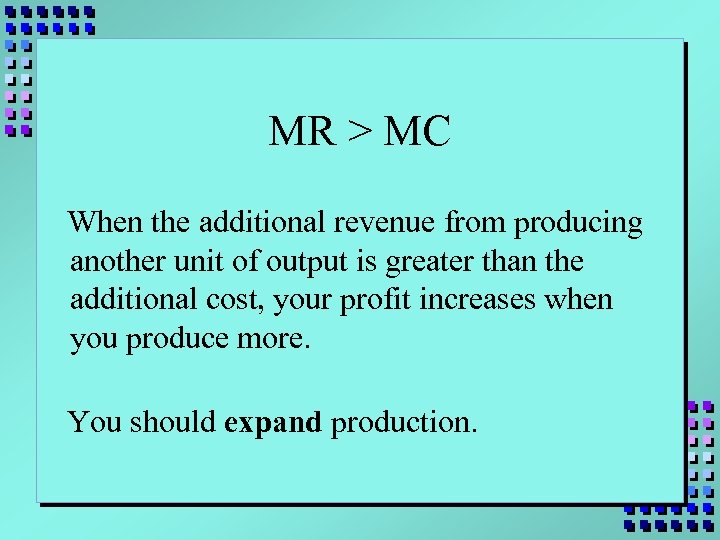MR > MC When the additional revenue from producing another unit of output is greater than the additional cost, your profit increases when you produce more. You should expand production.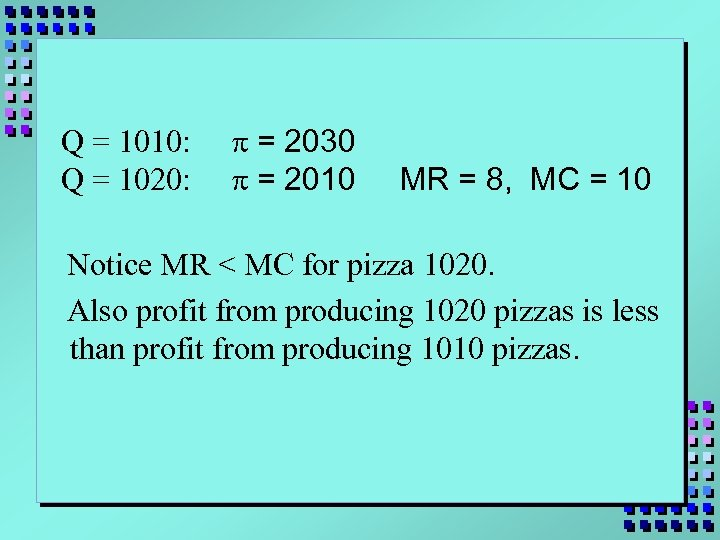Q = 1010: Q = 1020: p = 2030 p = 2010 MR = 8, MC = 10 Notice MR < MC for pizza 1020. Also profit from producing 1020 pizzas is less than profit from producing 1010 pizzas.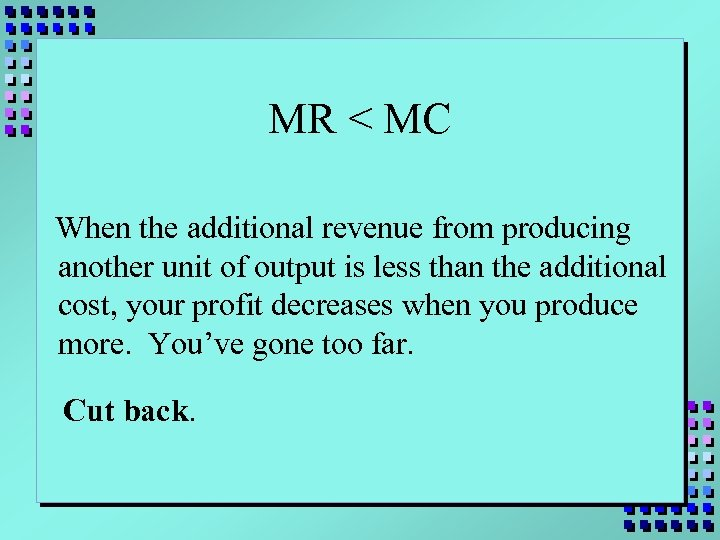MR < MC When the additional revenue from producing another unit of output is less than the additional cost, your profit decreases when you produce more. You’ve gone too far. Cut back.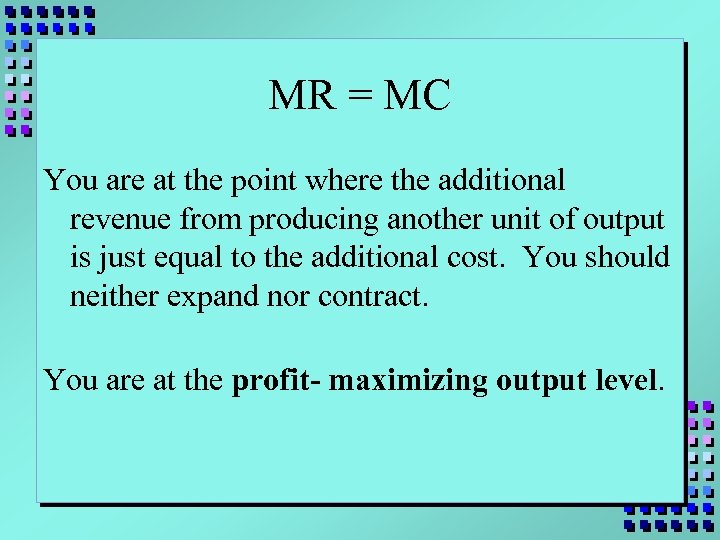MR = MC You are at the point where the additional revenue from producing another unit of output is just equal to the additional cost. You should neither expand nor contract. You are at the profit- maximizing output level.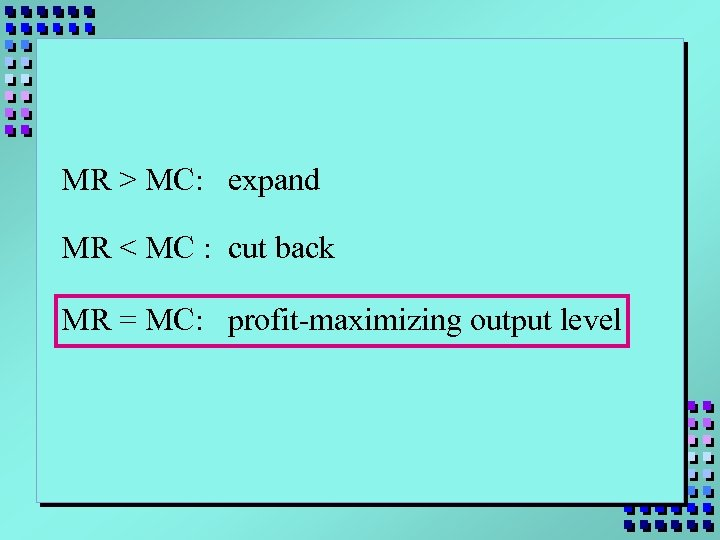MR > MC: expand MR < MC : cut back MR = MC: profit-maximizing output level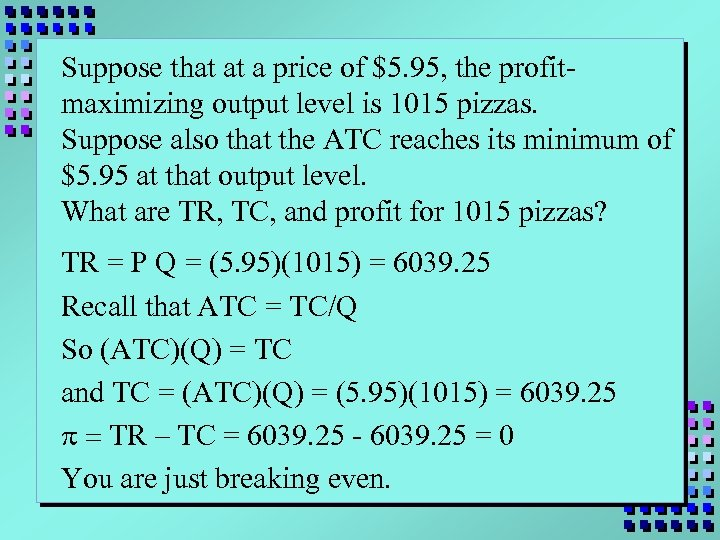Suppose that at a price of \$5. 95, the profitmaximizing output level is 1015 pizzas. Suppose also that the ATC reaches its minimum of \$5. 95 at that output level. What are TR, TC, and profit for 1015 pizzas? TR = P Q = (5. 95)(1015) = 6039. 25 Recall that ATC = TC/Q So (ATC)(Q) = TC and TC = (ATC)(Q) = (5. 95)(1015) = 6039. 25 p = TR – TC = 6039. 25 - 6039. 25 = 0 You are just breaking even.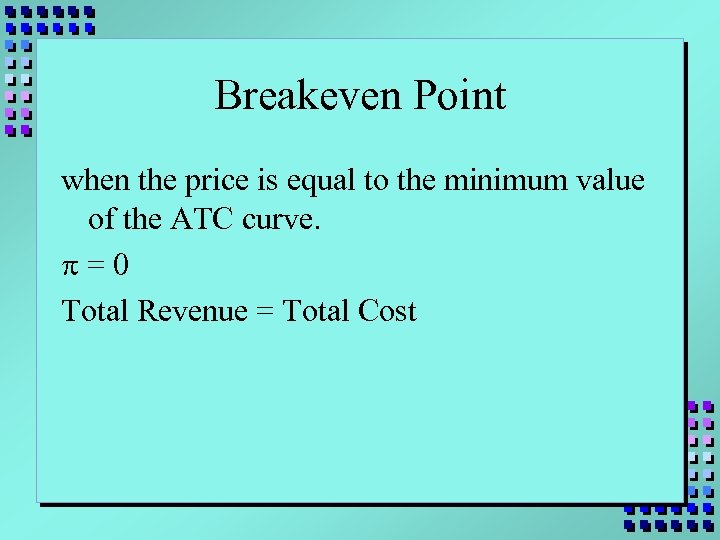Breakeven Point when the price is equal to the minimum value of the ATC curve. p=0 Total Revenue = Total Cost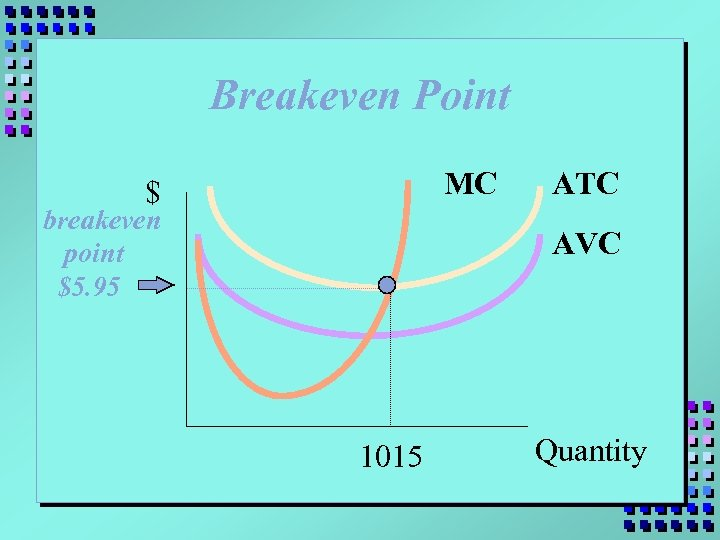Breakeven Point MC \$ breakeven point \$5. 95 ATC AVC 1015 Quantity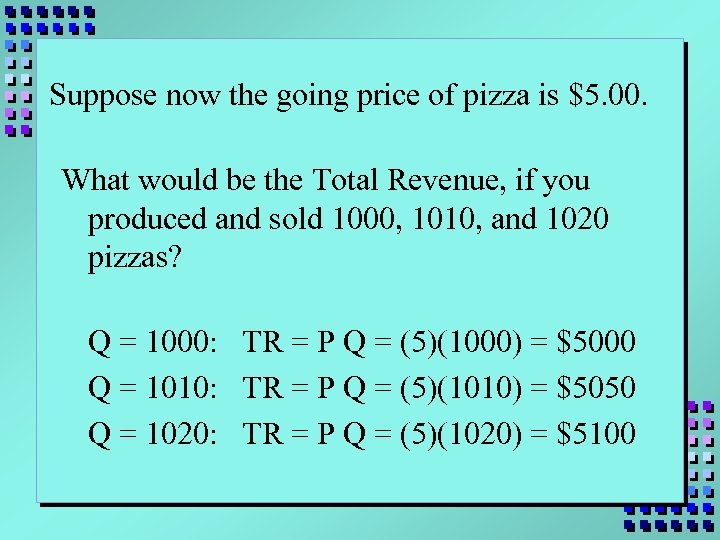Suppose now the going price of pizza is \$5. 00. What would be the Total Revenue, if you produced and sold 1000, 1010, and 1020 pizzas? Q = 1000: TR = P Q = (5)(1000) = \$5000 Q = 1010: TR = P Q = (5)(1010) = \$5050 Q = 1020: TR = P Q = (5)(1020) = \$5100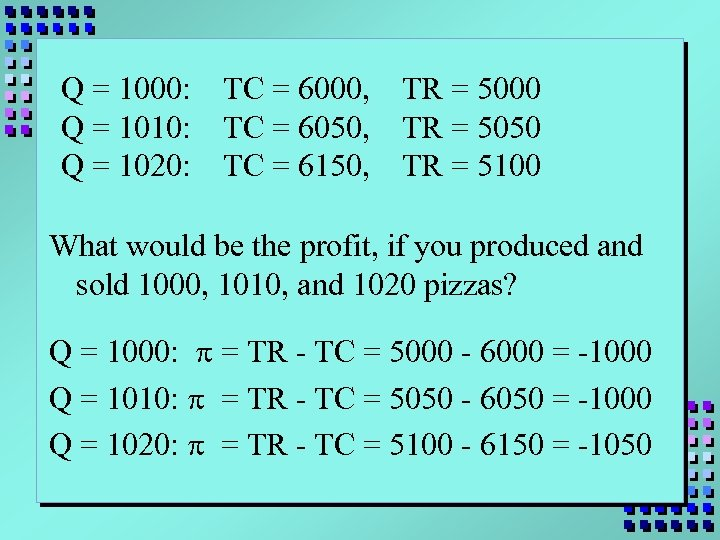Q = 1000: Q = 1010: Q = 1020: TC = 6000, TC = 6050, TC = 6150, TR = 5000 TR = 5050 TR = 5100 What would be the profit, if you produced and sold 1000, 1010, and 1020 pizzas? Q = 1000: p = TR - TC = 5000 - 6000 = -1000 Q = 1010: p = TR - TC = 5050 - 6050 = -1000 Q = 1020: p = TR - TC = 5100 - 6150 = -1050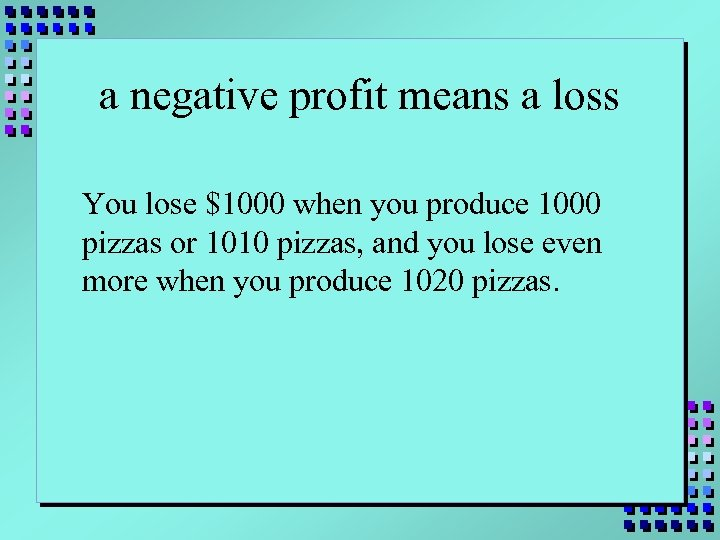a negative profit means a loss You lose \$1000 when you produce 1000 pizzas or 1010 pizzas, and you lose even more when you produce 1020 pizzas.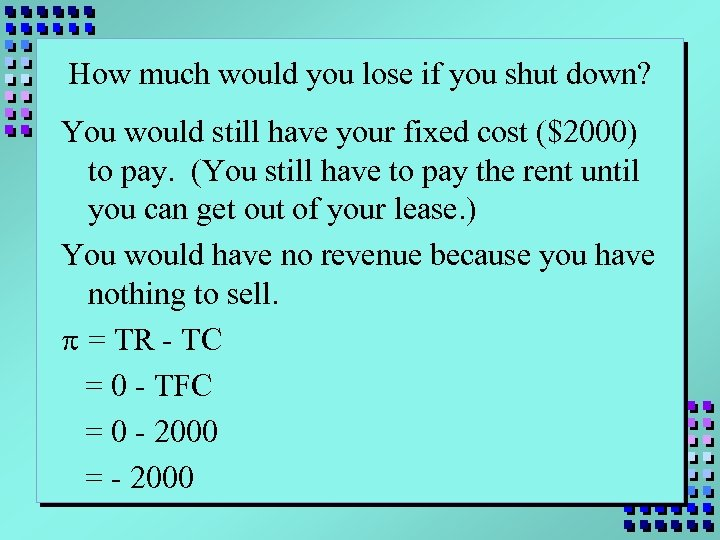How much would you lose if you shut down? You would still have your fixed cost (\$2000) to pay. (You still have to pay the rent until you can get out of your lease. ) You would have no revenue because you have nothing to sell. p = TR - TC = 0 - TFC = 0 - 2000 = - 2000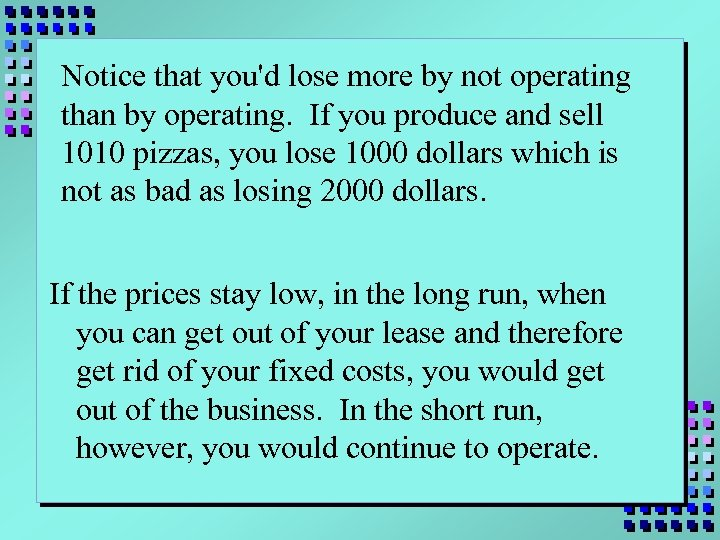Notice that you'd lose more by not operating than by operating. If you produce and sell 1010 pizzas, you lose 1000 dollars which is not as bad as losing 2000 dollars. If the prices stay low, in the long run, when you can get out of your lease and therefore get rid of your fixed costs, you would get out of the business. In the short run, however, you would continue to operate.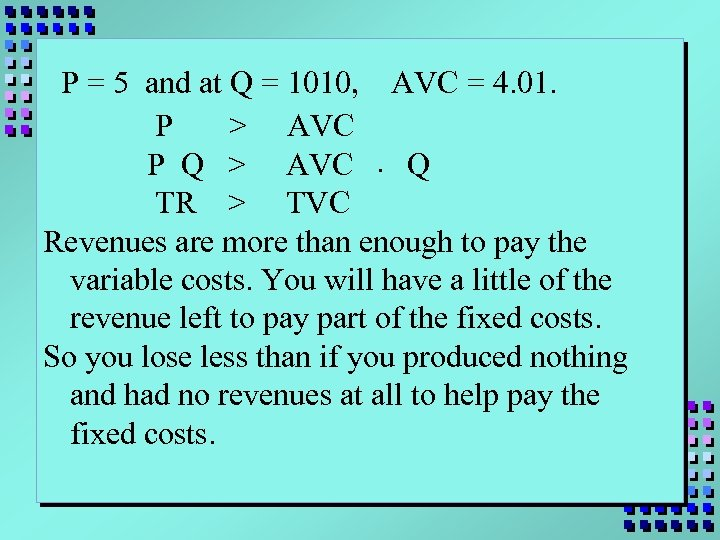P = 5 and at Q = 1010, AVC = 4. 01. P > AVC P Q > AVC. Q TR > TVC Revenues are more than enough to pay the variable costs. You will have a little of the revenue left to pay part of the fixed costs. So you lose less than if you produced nothing and had no revenues at all to help pay the fixed costs.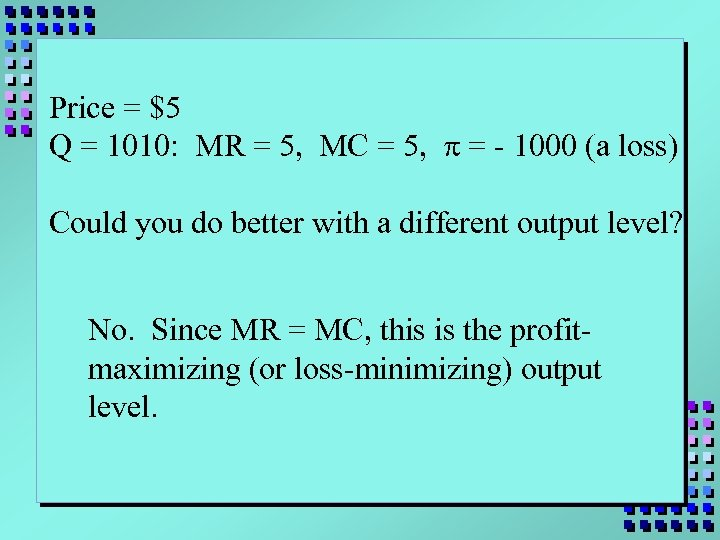Price = \$5 Q = 1010: MR = 5, MC = 5, p = - 1000 (a loss) Could you do better with a different output level? No. Since MR = MC, this is the profitmaximizing (or loss-minimizing) output level.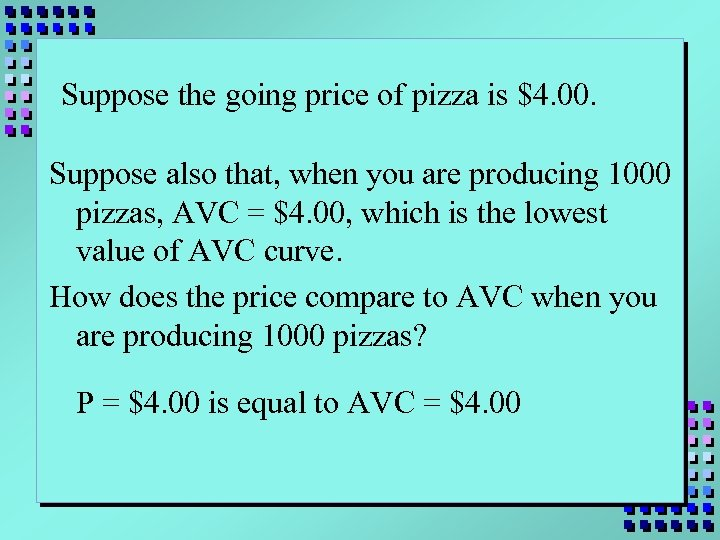Suppose the going price of pizza is \$4. 00. Suppose also that, when you are producing 1000 pizzas, AVC = \$4. 00, which is the lowest value of AVC curve. How does the price compare to AVC when you are producing 1000 pizzas? P = \$4. 00 is equal to AVC = \$4. 00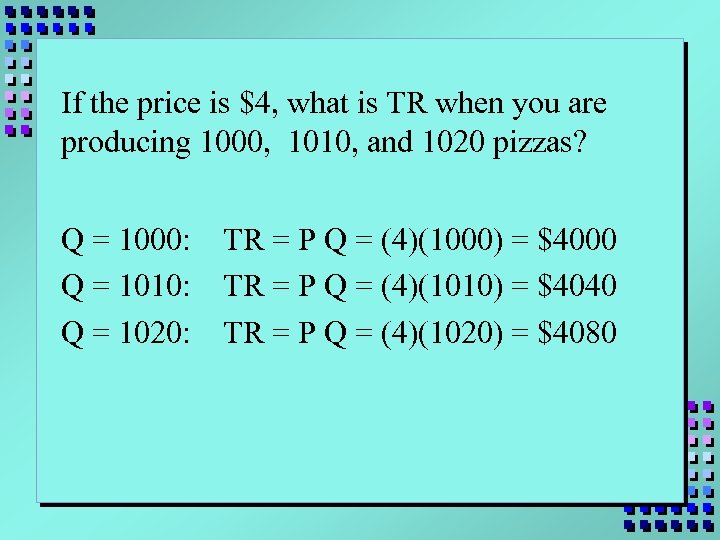If the price is \$4, what is TR when you are producing 1000, 1010, and 1020 pizzas? Q = 1000: Q = 1010: Q = 1020: TR = P Q = (4)(1000) = \$4000 TR = P Q = (4)(1010) = \$4040 TR = P Q = (4)(1020) = \$4080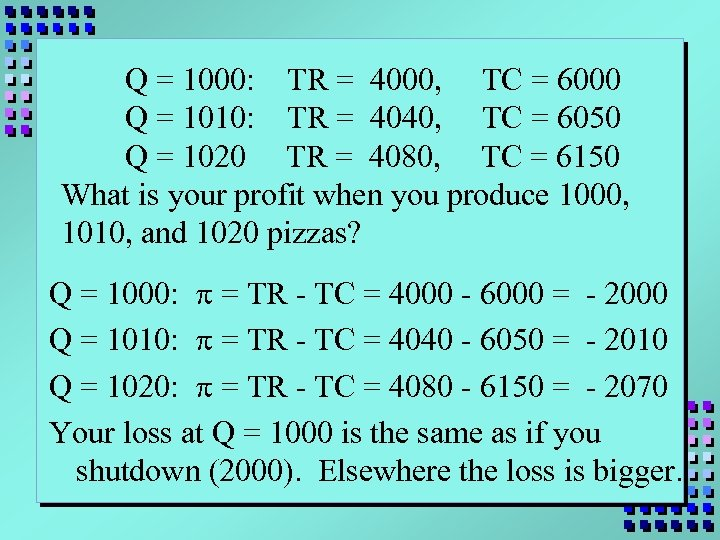Q = 1000: TR = 4000, TC = 6000 Q = 1010: TR = 4040, TC = 6050 Q = 1020 TR = 4080, TC = 6150 What is your profit when you produce 1000, 1010, and 1020 pizzas? Q = 1000: p = TR - TC = 4000 - 6000 = - 2000 Q = 1010: p = TR - TC = 4040 - 6050 = - 2010 Q = 1020: p = TR - TC = 4080 - 6150 = - 2070 Your loss at Q = 1000 is the same as if you shutdown (2000). Elsewhere the loss is bigger.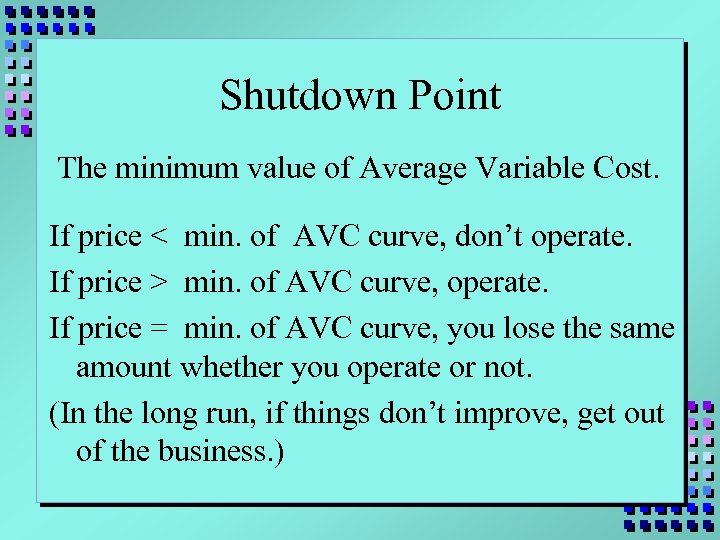Shutdown Point The minimum value of Average Variable Cost. If price < min. of AVC curve, don’t operate. If price > min. of AVC curve, operate. If price = min. of AVC curve, you lose the same amount whether you operate or not. (In the long run, if things don’t improve, get out of the business. )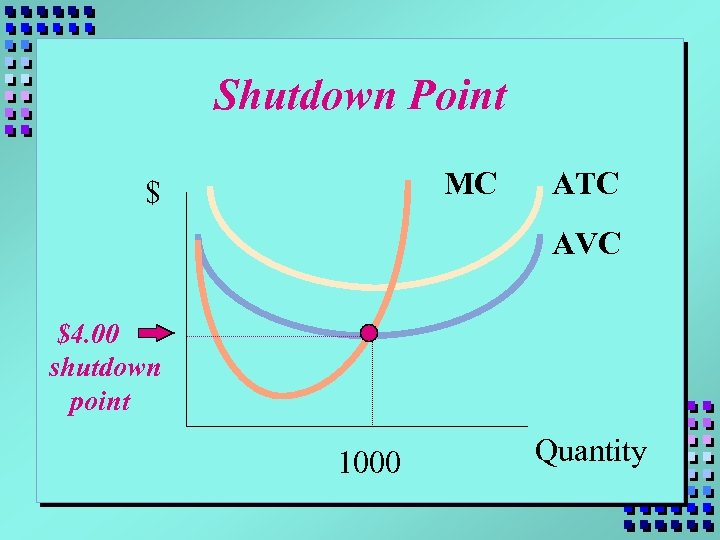Shutdown Point MC \$ ATC AVC \$4. 00 shutdown point 1000 Quantity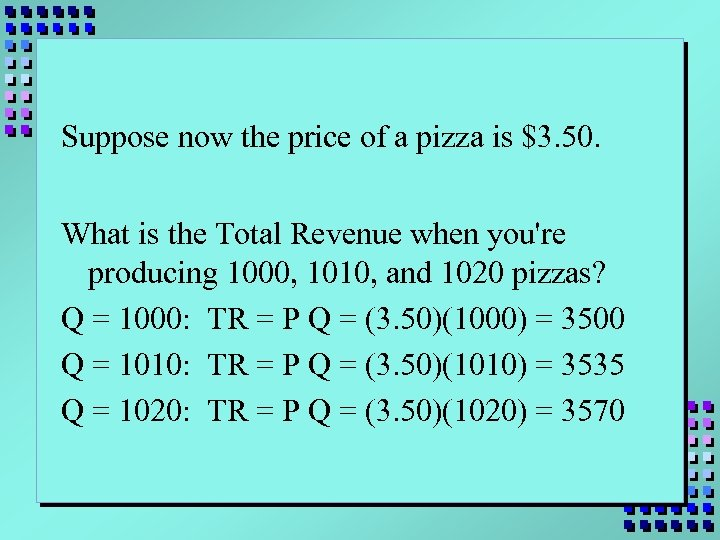Suppose now the price of a pizza is \$3. 50. What is the Total Revenue when you're producing 1000, 1010, and 1020 pizzas? Q = 1000: TR = P Q = (3. 50)(1000) = 3500 Q = 1010: TR = P Q = (3. 50)(1010) = 3535 Q = 1020: TR = P Q = (3. 50)(1020) = 3570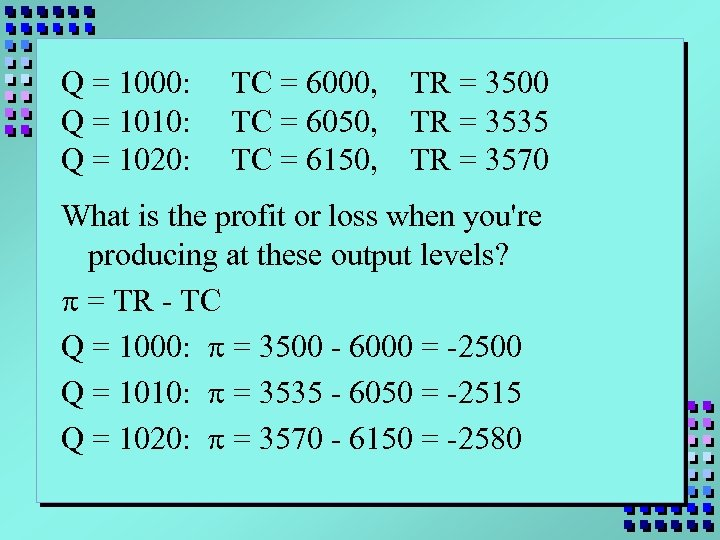Q = 1000: Q = 1010: Q = 1020: TC = 6000, TC = 6050, TC = 6150, TR = 3500 TR = 3535 TR = 3570 What is the profit or loss when you're producing at these output levels? p = TR - TC Q = 1000: p = 3500 - 6000 = -2500 Q = 1010: p = 3535 - 6050 = -2515 Q = 1020: p = 3570 - 6150 = -2580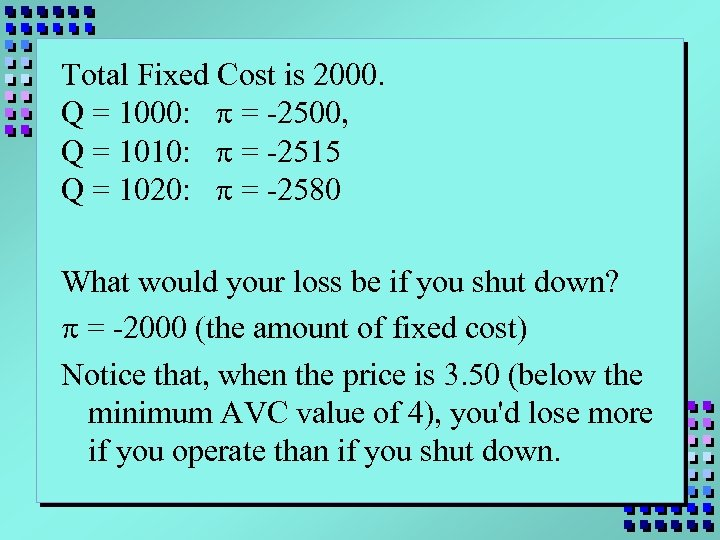Total Fixed Cost is 2000. Q = 1000: p = -2500, Q = 1010: p = -2515 Q = 1020: p = -2580 What would your loss be if you shut down? p = -2000 (the amount of fixed cost) Notice that, when the price is 3. 50 (below the minimum AVC value of 4), you'd lose more if you operate than if you shut down.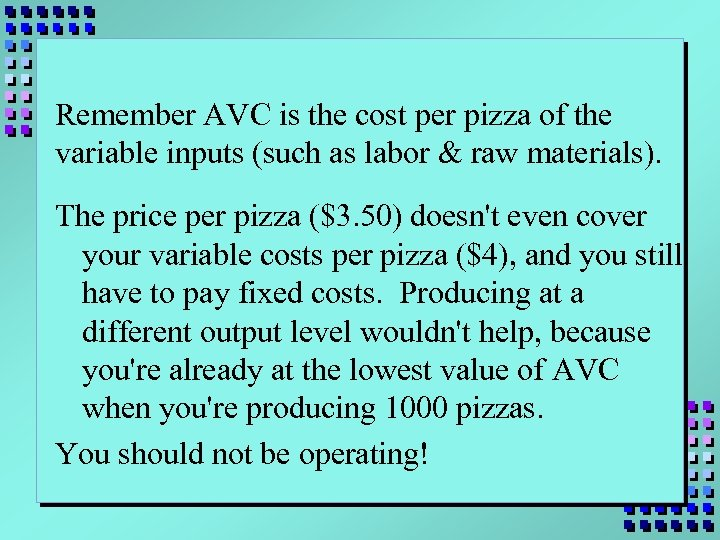Remember AVC is the cost per pizza of the variable inputs (such as labor & raw materials). The price per pizza (\$3. 50) doesn't even cover your variable costs per pizza (\$4), and you still have to pay fixed costs. Producing at a different output level wouldn't help, because you're already at the lowest value of AVC when you're producing 1000 pizzas. You should not be operating!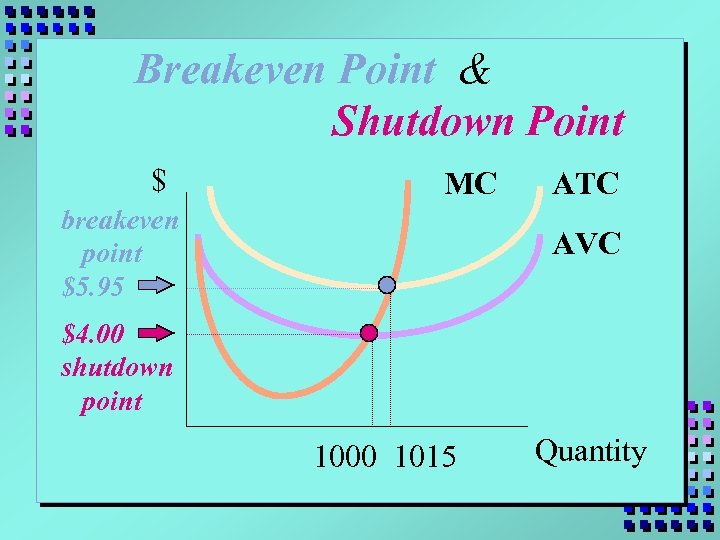Breakeven Point & Shutdown Point \$ MC breakeven point \$5. 95 ATC AVC \$4. 00 shutdown point 1000 1015 Quantity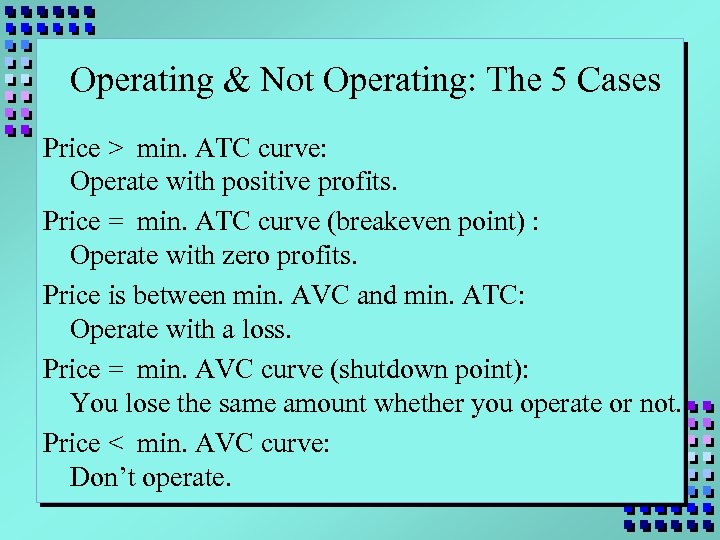Operating & Not Operating: The 5 Cases Price > min. ATC curve: Operate with positive profits. Price = min. ATC curve (breakeven point) : Operate with zero profits. Price is between min. AVC and min. ATC: Operate with a loss. Price = min. AVC curve (shutdown point): You lose the same amount whether you operate or not. Price < min. AVC curve: Don’t operate.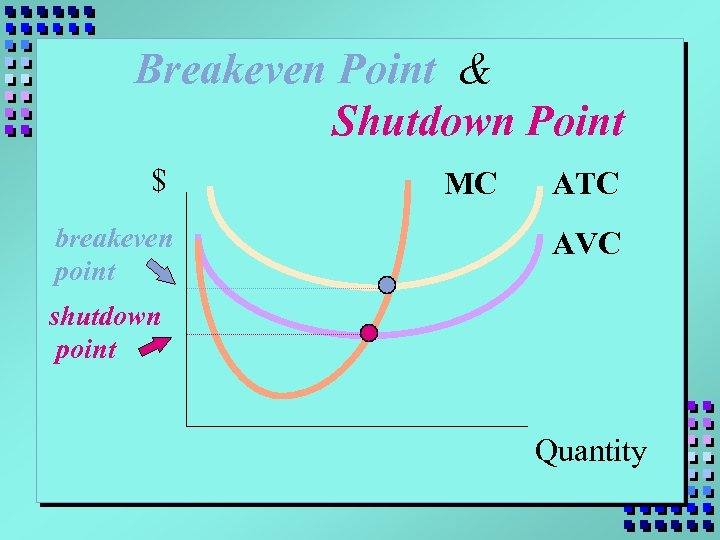Breakeven Point & Shutdown Point \$ breakeven point MC ATC AVC shutdown point Quantity